Most Affordable JEE | NEET | 8,9,10 Preparation by Kota's Top IITian Doctor Faculties

# NCERT Solutions for Class 11 Chemistry chapter 8 Redox Reactions PDF - eSaral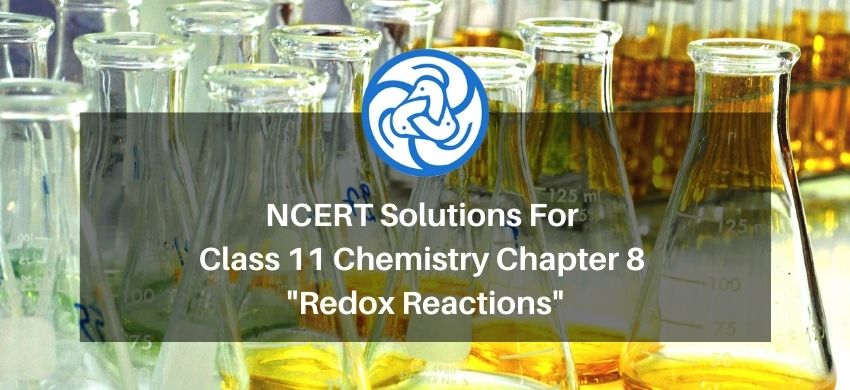# NCERT Solutions for Class 11 Chemistry chapter 8 Redox Reactions PDF

Hey, are you a class 11 student and looking for ways to download NCERT Solutions for Class 11 Chemistry chapter 8 Redox Reactions PDF? If yes. Then read this post till the end.

In this article, we have listed NCERT Solutions for Class 11 Chemistry chapter 8 Redox Reactions in PDF that are prepared by Kota’s top IITian’s Faculties by keeping Simplicity in mind.

If you want to learn and understand class 11 Chemistry chapter 8 "Redox Reactions" in an easy way then you can use these solutions PDF.

NCERT Solutions helps students to Practice important concepts of subjects easily. Class 11 Chemistry solutions provide detailed explanations of all the NCERT questions that students can use to clear their doubts instantly.

If you want to score high in your class 11 Chemistry Exam then it is very important for you to have a good knowledge of all the important topics, so to learn and practice those topics you can use eSaral NCERT Solutions.

So, without wasting more time Let’s start.

### Download The PDF of NCERT Solutions for Class 11 Chemistry Chapter 8 "Redox Reactions"

Question.1. Assign O.N. to the underlined elements in each of the following species:

(a) $\mathrm{NaH}_{2} \mathrm{PO}_{4}$

(b) $\mathrm{NaHSO}_{4}$

(c) $\mathrm{H}_{4} \mathrm{P}_{2} \mathrm{O}_{7}$

(d) $\mathrm{K}_{2} \mathrm{Mn} \mathrm{O}_{4}$

(e) $\mathrm{CaO}_{2}$

(f) $\mathrm{NaBH}_{4}$

(g) $\mathrm{H}_{2} \mathrm{~S}_{2} \mathrm{O}_{7}$

(h) $\mathrm{KAl}\left(\underline{\mathrm{S}} \mathrm{O}_{4}\right)_{2} .12 \mathrm{H}_{2} \mathrm{O}$

Solution. (a) $\mathrm{NaH}_{2} \mathrm{PO}_{4}$

Let the O.N. of $P=x$.

We know that,

The O.N. of $\mathrm{Na}=+1$

The O.N. of $\mathrm{H}=+1$

The O.N. of $0=-2$

Then, we have

$1(+1)+2(+1)+1(x)+4(-2)=0$

$1+2+x-8=0$

$\Rightarrow \mathrm{x}=+5$

Hence, the O.N. of $P$ is $+5$

(b) $\mathrm{NaHSO}_{4}$

Let the O.N. of $S=\mathrm{x}$.

We know that,

The O.N. of $\mathrm{Na}=+1$

The O.N. of $\mathrm{H}=+1$

The O.N. of $O=-2$

$1(+1)+1(+1)+1(x)+4(-2)=0$

$\Rightarrow 1+1+x-8=0$

$\Rightarrow \mathrm{x}=+6$

Hence, the O.N. of $S$ is $+6$.

(c) $\mathrm{H}_{4} \mathrm{P}_{2} \mathrm{O}_{7}$

Let the O.N. of $\mathrm{P}=\mathrm{x}$

We know that,

The O.N. of $\mathrm{H}=+1$

The O.N. of $0=-2$

Then, we have

$4(+1)+2(x)+7(-2)=0$

$\Rightarrow 4+2 x-14=0$

$\Rightarrow 2 \mathrm{x}=+10$

$\Rightarrow x=+5$

Hence, the O.N. of $\mathrm{P}$ is $+5$

(d) $\mathrm{K}_{2} \mathrm{MnO}_{4}$

Let the O.N. of $M n=x$.

We know that,

The O.N. of $K=+1$

The O.N. of $0=-2$

Then, we have

$2(+1)+x+4(-2)=0$

$\Rightarrow x=+6$

Hence, the O.N. of $\mathrm{Mn}$ is $+6$

(e) $\mathrm{Ca} \underline{\mathrm{O}}_{2}$

Let the O.N. of $O=\mathrm{x}$.

We know that,

The O.N. of $\mathrm{Ca}=+2$

Then, we have

$(+2)+2(x)+0$

$\Rightarrow 2+2 x=0$

$\Rightarrow \mathrm{x}=-1$

$\Rightarrow \mathrm{x}=-1$

Hence, the O.N. of 0 is $-1$

(f) $\mathrm{NaBH}_{4}$

Let the O.N. of $B=x$.

We know that,

The O.N. of $\mathrm{Na}=+1$

The O.N. of $\mathrm{H}=+1$

Then, we have

$1(+1)+1(x)+4(-1)=0$

$\Rightarrow 1+x-4=0$

$\Rightarrow x=-3$

Hence, the O.N. of $B$ is $+3$

(g) $\mathrm{H}_{2} \underline{\mathrm{S}}_{2} \mathrm{O}_{7}$

Let the O.N. of $S=x$.

We know that,

The O.N. of $\mathrm{H}=+1$

The O.N. of $0=-2$

Then, we have

$2(+1)+2(x)+7(-2)=0$

$\Rightarrow 2+2 x-14=0$

$\Rightarrow 2+2 \mathrm{x}-14=0$

$\Rightarrow 2 \mathrm{x}=12$

Hence, the O.N. of $S$ is $+6$

(h) $\operatorname{KAl}\left(\underline{\mathrm{S}} \mathrm{O}_{4}\right)_{2} \cdot 12 \mathrm{H}_{2} \mathrm{O}$

Let the O.N. of $S=\mathrm{x}$.

We know that,

The O.N. of $\mathrm{K}=+1$

The O.N. of $\mathrm{Al}=+3$

The O.N. of $\mathrm{Al}=+3$

The O.N. of $O=-2$

The O.N. of $\mathrm{H}=+1$

Then, we have

$1(+1)+1(+3)+2 x+8(-2)$

$+24(+1)+12(-2)=0$

$\Rightarrow 1+3+2 x-16+24-24=0$

$\Rightarrow \mathrm{x}=+6$

Hence, the O.N. of $S$ is $+6$

$\mathrm{Or}$

We can ignore the water molecule as it is a neutral molecule. Then, the sum of the O.N.s of all atoms of the water molecule may be taken as zero. Therefore, after ignoring the water molecule, we have

Let the O.N. of $S=\mathrm{x}$.

We know that,

The O.N. of $\mathrm{K}=+1$

The O.N. of $0=-2$

The O.N. of $A l=+3$

The O.N. of $\mathrm{H}_{2} \mathrm{O}=0$

Then, we have

$1(+1)+1(+3)+2 x+8(-2)=0$

$\Rightarrow 1+3+2 x-16=0$

$\Rightarrow \mathrm{x}=+6$

Hence, the O.N. of $S$ is $+6$

Question 2. What are the O.N. of the underlined elements in each of the following and how do you rationalise your results?

(a) $\mathrm{KI}_{3}$

(b) $\mathrm{H}_{2} \underline{\mathrm{S}}_{4} \mathrm{O}_{6}$

(c) $\underline{\mathrm{Fe}}_{3} \mathrm{O}_{4}$

(d) $\underline{\mathrm{CH}}_{3} \underline{\mathrm{C}} \mathrm{H}_{2} \mathrm{OH}$

(e) $\underline{\mathrm{C}} \mathrm{H}_{3} \underline{\mathrm{C}} \mathrm{OOH}$

Solution: (a) $\mathrm{KI}_{3}$

Let the O.N. of $S=x$.

We know that.

the O.N. (O.N.) of $\mathrm{K}=+1$

Hence, the average O.N. of I

$1(+1)+3(x)=0$

$\Rightarrow 1+3 x=0$

$\Rightarrow \mathrm{x}=-\frac{1}{3}$

However, $0 . \mathrm{N}$. cannot be fractional. Therefore, we will have to consider the structure of $K I_{3}$ to find the oxidation states.

In a $\mathrm{KI}_{3}$, molecule, an atom of iodine forms a coordinate covalent bond with an iodine molecule.

$\stackrel{+1}{\mathrm{~K}}^{+}\left[\stackrel{0}{\mathrm{I}}-\stackrel{0}{\mathrm{I}} \leftarrow \mathrm{I}^{-1}\right]^{-}$

Hence, in a $\mathrm{KI}_{3}$ molecule, the $0 . \mathrm{N}$. of the two $\mathrm{I}$ atoms forming the $\mathrm{I}_{2}$ molecule is 0 , whereas the $0 . \mathrm{N}$. of the I atom forming the coordinate bond is $-1$.

(b) $\mathrm{H}_{2} \underline{\mathrm{S}}_{4} \mathrm{O}_{6}$

Let the O.N. of $S=\mathrm{x}$.

We know that,

The O.N. of $\mathrm{H}=+1$

The O.N. of $\mathrm{O}=-2$

Now, $2(+1)+4(x)+6(-2)=0$

$\Rightarrow 2+4 x-12=0$

$\Rightarrow 4 \mathrm{x}=10$

$\Rightarrow \mathrm{x}=+2.5$

However, O. N. cannot be fractional. Hence, S must be present in different oxidation states in the molecule.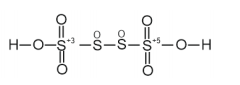The 0. N. of two of the four $S$ atoms is $+5$ and the $0 . N$. of the other two $S$ atoms is 0 .

(c) $\mathrm{Fe}_{3} \mathrm{O}_{4}$

Let the O.N. of $F e=x$.

We know that,

The O.N. of $O=-2$

Now, $3(x)+4(-2)=0$

$\Rightarrow 3 x-8=0$

$\Rightarrow 3 x=8$

$\Rightarrow \mathrm{x}=+2.5$

the $0 .$ N. of $\mathrm{Fe}$ is found to be $+2.5$. However, $0 .$ N. cannot be fractional.

$\mathrm{Fe}_{3} \mathrm{O}_{4}$ is mixed oxide is has $\mathrm{Fe} 0$ and $\mathrm{Fe}_{2} \mathrm{O}_{3}$,

the oxidation state of Fe in FeO:

$x+1(-2)=0$

$\mathrm{x}=2$

the oxidation state of $\mathrm{Fe}$ in $\mathrm{Fe}_{2} \mathrm{O}_{3}$ :

$2 x+3(-2)=0$

$2 x+3(-2)=0$

$\mathrm{x}=3$

the 0. N. of Fe is found to be 2 and 3 in $\mathrm{FeO}$ and $\mathrm{Fe}_{2} \mathrm{O}_{3}$ respectively.

(d) $\quad \mathrm{CH}_{3} \underline{\mathrm{CH}}_{2} \mathrm{OH}$

Let the O.N. of $C=x$.

We know that,

The O.N. of $\mathrm{H}=+1$

The O.N. of $0=-2$

Now,

$2(x)+6(+1)+1(-2)=0$

$\Rightarrow 2 x+6-2=0$

$\Rightarrow x=-2$

Hence, the O. N. of $\mathrm{C}$ is $-2$

(e) $\quad \mathrm{CH}_{3} \underline{\mathrm{COOH}}$

Let the O.N. of $C=\mathrm{x}$.

We know that,

The O.N. of $\mathrm{H}=+1$

The O.N. of $O=-2$

Now, $2(x)+4(1)+2(-2)=0$

$\Rightarrow 2 x+4-4=0$

$\Rightarrow \mathrm{x}=0$

However, 0 is average $0 . \mathrm{N}$. of $\mathrm{C}$. The two carbon atoms present in this molecule are present in different environments. Hence, they cannot have the same O.N..

Thus, $\mathrm{C}$ exhibits the oxidation states of $+2$ and $-2$ in $\mathrm{CH}_{3} \mathrm{COOH}$.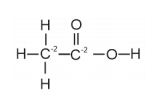Question. 3. Justify that the following reactions are redox reactions:

(a)$\mathrm{CuO}(\mathrm{s})+\mathrm{H}_{2}(\mathrm{~g})+\mathrm{Cu}(\mathrm{s})+\mathrm{H}_{2} \mathrm{O}(\mathrm{g})$

(b) $\mathrm{Fe}_{2} \mathrm{O}_{3}(\mathrm{~s})+3 \mathrm{CO}(\mathrm{g}) \rightarrow 2 \mathrm{Fe}(\mathrm{s})+3 \mathrm{CO}_{2}(\mathrm{~g})$

(c) $4 \mathrm{BCl}_{3}(\mathrm{~g})+3 \mathrm{LiAlH}_{4}(\mathrm{~s}) \rightarrow 2 \mathrm{~B}_{2} \mathrm{H}_{6}(\mathrm{~g})+3 \mathrm{LiCl}(\mathrm{s})+3 \mathrm{AlCl}_{3}(\mathrm{~s})$

(d) $2 \mathrm{~K}(\mathrm{~s})+\mathrm{F}_{2}(\mathrm{~g}) \rightarrow 2 \mathrm{~K}^{+} \mathrm{F}^{-}(\mathrm{s})$

(e) $4 \mathrm{NH}_{3}+5 \mathrm{O}_{2}(\mathrm{~g}) \rightarrow 4 \mathrm{NO}(\mathrm{g})+6 \mathrm{H}_{2} \mathrm{O}(\mathrm{g})$

Solution:(a)$\mathrm{CuO}(\mathrm{s})+\mathrm{H}_{2}(\mathrm{~g})+\mathrm{Cu}(\mathrm{s})+\mathrm{H}_{2} \mathrm{O}(\mathrm{g})$

Let us write the O.N. of each element involved in the given reaction as:

$\mathrm{Cu} \mathrm{O}(\mathrm{s})+\mathrm{H}_{2}(\mathrm{~g}) \rightarrow \mathrm{Cu}(\mathrm{s})+\mathrm{H}_{2} \mathrm{O}(\mathrm{g})$

Here,

$2 e^{-}+C u^{+2} \rightarrow C u$

the O.N. of Cu decreases from $+2$ in CuO to 0 in Cu, i.e., $\mathrm{CuO}$ is reduced to Cu. Or in the above reaction addition of electron is taking place so it is a reduction.

$\stackrel{0}{\mathrm{H}_{2}}(\mathrm{~g}) \rightarrow \mathrm{Cu}(\mathrm{s})+\mathrm{H}_{2} \mathrm{O}$

Also, the O.N. of $\mathrm{H}$ increases from zero in $\mathrm{H}_{2}$ to $+1$ in $\mathrm{H}_{2} \mathrm{O}$ i.e., $\mathrm{H}_{2}$ is oxidized to $\mathrm{H}_{2} \mathrm{O}$

In the above reaction oxidation and reduction, both is taking place simultaneously Hence, this reaction is a redox reaction.

(b) $\mathrm{Fe}_{2} \mathrm{O}_{3}(\mathrm{~s})+3 \mathrm{CO}(\mathrm{g}) \rightarrow 2 \mathrm{Fe}(\mathrm{s})+3 \mathrm{CO}_{2}(\mathrm{~g})$

Let us write the O.N. of each element in the given reaction as:

$\stackrel{+3}{\mathrm{~F}} \mathrm{e}_{2} \mathrm{O}_{3}(\mathrm{~s})+3 \stackrel{+2-2}{\mathrm{C}} \mathrm{O}(\mathrm{g}) \rightarrow 2 \mathrm{Fe}(\mathrm{s})+3 \stackrel{+4-2}{\mathrm{C}} \mathrm{O}_{2}(\mathrm{~g})$

$F e^{+3}+3 e^{-} \rightarrow F e$

Here, the O.N. of Fe decreases from $+3$ in $\mathrm{Fe}_{2} \mathrm{O}_{3}$ to zero in Fe or addition of electron involved so $\mathrm{Fe}_{2} \mathrm{O}_{3}$ is reduced to $\mathrm{Fe}$.

$\stackrel{+2-2}{C} 0(g) \rightarrow \stackrel{+4-2}{C} \mathrm{O}_{2}(\mathrm{~g})$ the $\mathrm{O} . \mathrm{N} .$ of $\mathrm{C}$ increases from $+2$ in $\mathrm{C} 0$ to $+4$ in $\mathrm{CO}_{2}$ i.e., CO is oxidized to $\mathrm{CO}_{2}$.

In the above reaction oxidation and reduction, both is taking place simultaneously Hence, this reaction is a redox reaction.

(c) $\quad 4 \mathrm{BCl}_{3}(\mathrm{~g})+3 \mathrm{LiAlH}_{4}(\mathrm{~s}) \rightarrow 2 \mathrm{~B}_{2} \mathrm{H}_{6}(\mathrm{~g})+3 \mathrm{LiCl}(\mathrm{s})+3 \mathrm{AlCl}_{3}(\mathrm{~s})$

The O.N. of each element in the given reaction can be represented as:

$4 \mathrm{~B} \mathrm{Cl}_{3}(\mathrm{~g})+3 \mathrm{LiAlH}_{4}(\mathrm{~s}) \rightarrow 2 \mathrm{~B}_{2}^{-3} \mathrm{H}_{6}(\mathrm{~g})$

$+3 \mathrm{LiCl}(\mathrm{s})+3 \mathrm{AlCl}_{3}(\mathrm{~s})$

In this reaction, the O.N. of B decreases from $+3$ in $\mathrm{BCl}_{3}$ to $-3$ in $\mathrm{B}_{2} \mathrm{H}_{6}$.

i.e., $\mathrm{BCl}_{3}$ is reduced $\operatorname{toB}_{2} \mathrm{H}_{6}$. Also, the O.N. of $\mathrm{H}$ increases from $-1$ in $\mathrm{LiAlH}_{4}$ to $+1$ in $\mathrm{B}_{2} \mathrm{H}_{6}$ i.e., $\mathrm{LiAl} \mathrm{H}_{4}$ is oxidized to $\mathrm{B}_{2} \mathrm{H}_{6}$. In the above reaction oxidation and reduction, both is taking place simultaneously Hence, this reaction is a redox reaction.

(d) $2 \mathrm{~K}(\mathrm{~s})+\mathrm{F}_{2}(\mathrm{~g}) \rightarrow 2 \mathrm{~K}^{+} \mathrm{F}^{-}(\mathrm{s})$

The O.N. of each element in the given reaction can be represented as: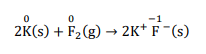In this reaction, the O.N. of $K$ increases from zero in $K$ to $+1$ in $\mathrm{KF}$. i.e., $K$ is oxidised to $\mathrm{KF}$. Also, the O.N. of $F_{2}$ decreases from 0 in $F_{2}$ and $-$ 1 in $K F$ i.e.redution is occure.

(e) $4 \mathrm{NH}_{3}+50_{2}(\mathrm{~g}) \rightarrow 4 \mathrm{NO}(\mathrm{g})+6 \mathrm{H}_{2} \mathrm{O}(\mathrm{g})$

The O.N. of each element in the given reaction can be represented as:

$4 \mathrm{~N}^{-3+1} \mathrm{H}_{3}+5 \mathrm{O}_{2}(\mathrm{~g}) \rightarrow 4 \mathrm{~N}^{+2-2}(\mathrm{~g})$

$+6 \mathrm{H}_{2}^{+1} \mathrm{O}(\mathrm{g})$

Here, the O.N. of $N$ increases from - 3 in $\mathrm{NH}_{3}$ to $+2$ in NO. so oxidation is taking place there

the O.N. of $\mathrm{O}_{2}$ decreases from 0 in $\mathrm{O}_{2}$ to $-2$ in $\mathrm{NO}$ and $\mathrm{H}_{2} \mathrm{O}$ i.e., so the gain of electron is occur here so $\mathrm{O}_{2}$ is reduced.

In the above reaction oxidation and reduction, both is taking place simultaneously. Hence, this reaction is a redox reaction.

Question4. Fluorine reacts with ice and results in the change:

$\mathrm{H}_{2} \mathrm{O}(\mathrm{s})+\mathrm{F}_{2}(\mathrm{~g}) \rightarrow \mathrm{HF}(\mathrm{g})+\mathrm{HOF}(\mathrm{g})$

Justify that this reaction is a redox reaction.

Solution. Let us write the O.N. of each atom involved in the given reaction above its symbol as: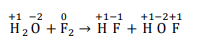Here, we have observed that the O.N. of $F$ increases from 0 in $\mathrm{F}_{2}$ to $+1$ in HOF.

Also, the O.N. of fluorine decreases from 0 in $\mathrm{F}_{2}$ to $-1$ in HF. Thus, in the above reaction, $F$ is both oxidized and reduced. Hence, the given reaction is a redox reaction.

Quesition 5. Calculate the O.N. of sulphur, chromium and nitrogen in $\mathrm{H}_{2} \mathrm{SO}_{5}, \mathrm{Cr}_{2} \mathrm{O}_{7}^{2-}$ and $\mathrm{NO}_{3}^{-}$. Suggest structure of these compounds. Count for the fallacy.

Solution. (i) average O.N. of sulphure in $\mathrm{H}_{2} \mathrm{SO}_{5}$

Let the O.N. of $S=x$.

We know that,

The O.N. of $O=-2$

The O.N. of $\mathrm{H}=+1$

Now,

$2(+1)+1(x)+5(-2)=0$

$\Rightarrow 2+x-10=0$

$\Rightarrow \mathrm{x}=+8$

However, the O.N. of $S$ cannot be $+8 . \mathrm{S}$ has six valence electrons. Therefore, the $0 . \mathrm{N}$. of $S$ cannot be more than $+6$.

The structure of $\mathrm{H}_{2} \mathrm{SO}_{5}$ is shown as follows: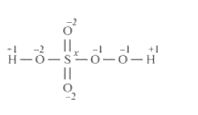Now, $2(+1)+1(\mathrm{x})+3(-2)+2(-1)=0$

$\Rightarrow 2+x-6-2=0$

$\Rightarrow x=+6$

Therefore, the O. N. of $S$ is $+6$.

(ii) $\mathrm{Cr}_{2} \mathrm{O}_{7}^{2-}$

Let the O.N. of $C r=\mathrm{x}$.

We know that,

The O.N. of $0=-2$

$2(x)+7(-2)=-2$

$\Rightarrow 2 \mathrm{x}-14=-2$

$\Rightarrow \mathrm{x}=+6$

Here, there is no fallacy about the 0. N. of $\mathrm{Cr}$ in $\mathrm{Cr}_{2} \mathrm{O}_{7}^{2-}$

The structure of $\mathrm{Cr}_{2} \mathrm{O}_{7}^{2-}$ is shown as follows: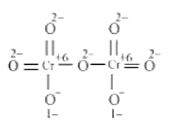Here, each of the two Cr atoms exhibits the O. N. of $+6$.

(iii) $\mathrm{NO}_{3}^{-}$.

Let the O.N. of $N=x$.

We know that,

The O.N. of $O=-2$

$1(x)+3(-2)=-1$

$\Rightarrow x-6=-1$

$\Rightarrow \mathrm{x}=+5$

Here, there is no fallacy about the O.N. of $\mathrm{N}$ in $\mathrm{NO}_{3}^{-}$

The structure of $\mathrm{NO}_{3}^{-}$ is shown as follows: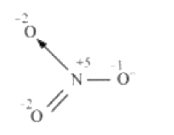The $\mathrm{N}$ atom exhibits the $0 . \mathrm{N}$. of $+5$.

Question 6. Write formulas for the following compounds:

(a) $\quad$ Mercury(II) chloride

(b) Nickel(II) sulphate

(c) Tin(IV) oxide

(d) Thallium(I) sulphate

(e) Iron(III) sulphate

(f) Chromium(III) oxide

Solution. (a) Mercury(II) chloride: Mercury(II) $=H g^{+2}$ and chloride $=C l^{-}$ so the formula of a compound is $\mathrm{HgCl}_{2}$

(b) Nickel(II) sulphate: nickel $(\mathrm{II})=\mathrm{N} i^{+2}$ and sulphate $=S O_{4}^{-2}$ so the formula of a compound is $\mathrm{NiSO}_{4}$

(c) $\quad$ Tin(IV) oxide: $\operatorname{Tin}(I V)=S n^{+4}+$ and oxide $=0^{-2}$ so the formula of a compound is $\operatorname{SnO}_{2}$

(d) Thallium(I) sulphate:

Thallium(I) $=\mathrm{Tl}^{+1}$

sulphate $=S O_{4}^{-2}$

the formula of a compound is $\mathrm{TI}_{2} \mathrm{SO}_{4}$

(e) Iron(III) sulphate: Iron(III) $=F e^{+3}$ $+$ and sulphate $=$

$\mathrm{SO}_{4}^{-2}$ so the formula of a compound is $\mathrm{Fe}_{2}\left(\mathrm{SO}_{4}\right)_{3}$

(f) Chromium(III) oxide: Chromium(III) $=C r^{+3}+$ and oxide

$=0^{-2}$ so the formula of a compound is $\mathrm{Cr}_{2} \mathrm{O}_{3}$

Question 7. Suggest a list of the substances where carbon can exhibit oxidation states from $-4$ to $+4$ and nitrogen from $-3$ to $+5$.

Solution: The substances where carbon can exhibit oxidation states from $-4$ to $+4$ are listed in the following table.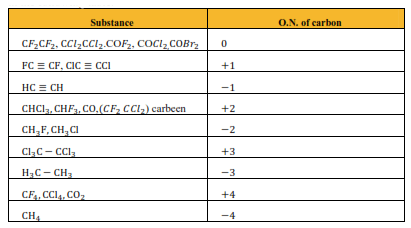The substances where nitrogen can exhibit oxidation states from $-3$ to $+5$ are listed in the following table.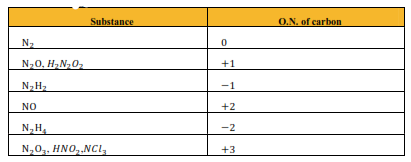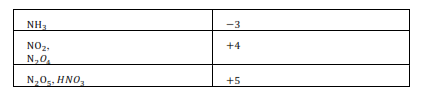Question. 8. While sulphur dioxide and hydrogen peroxide, ozone can act as oxidising as well as reducing agents in their reactions, nitric acid act only as oxidants. Why?

Solution. In sulphur dioxide $\left(\mathrm{SO}_{2}\right)$, the O.N. (O. N.) of $\mathrm{S}$ is $+4$ and the range of the $0 . \mathrm{N}$. that $\mathrm{S}$ can have is from $+6$ to $-2$.Therefore, $\mathrm{SO}_{2}$ can act as an oxidising as well as a reducing agent.

$\mathrm{SO}_{2} \rightarrow \mathrm{SO}_{3}$ (oxidation)

$\mathrm{SO}_{2} \rightarrow \mathrm{S}^{-2}$ (reduction)

In hydrogen peroxide $\left(\mathrm{H}_{2} \mathrm{O}_{2}\right)$ the $0 . \mathrm{N}$. of 0 is $-1$ and the range of the $0 . \mathrm{N}$. that $\mathrm{O}$ can have is from 0 to $-2.0$ can sometimes

also attain the O.N.s $+1$ and $+2$. Hence, $\mathrm{H}_{2} \mathrm{O}_{2}$ can act as an oxidising as well as a reducing agent.

$\mathrm{H}_{2} \mathrm{O}_{2} \rightarrow \mathrm{O}_{2}$ (oxidation)

$\mathrm{H}_{2} \mathrm{O}_{2} \rightarrow \mathrm{H}_{2} \mathrm{O}$ (reduction)

In ozone, the $0 . \mathrm{N}$. of Oxygen is zero and the range of the $0 . \mathrm{N}$. that $\mathrm{O}$ can have is from $+2$ to $-2$.

Therefore, the $0 . \mathrm{N}$. of 0 can only decrease in this case. Hence, $0_{3}$ acts as an oxidant.

$\mathrm{O}_{3} \rightarrow \mathrm{O}_{2}+[\mathrm{O}]$

Ozone reduces peroxides to oxides and in turn gets reduced to oxygen so used as reductant

In nitric acid $\left(\mathrm{HNO}_{3}\right)$, the $0 . \mathrm{N}$. of $\mathrm{N}$ is $+5$ and the range of the $0 . \mathrm{N}$. that $\mathrm{N}$ can have is from $+5$ to $-3$. Therefore, the 0.N. of $\mathrm{N}$ can only decrease in this case. Hence, $\mathrm{HNO}_{3}$ acts only as an oxidant.

Question. $9 .$ Consider the reactions:

(a) $6 \mathrm{CO}_{2}(\mathrm{~g})+6 \mathrm{H}_{2} \mathrm{O}(\mathrm{l})$

$\rightarrow \mathrm{C}_{6} \mathrm{H}_{12} \mathrm{O}_{6}(\mathrm{aq})+6 \mathrm{O}_{2}(\mathrm{~g})$

(b) $\mathrm{O}_{3}(\mathrm{~g})+\mathrm{H}_{2} \mathrm{O}_{2}(\mathrm{l})$

$\rightarrow \mathrm{H}_{2} \mathrm{O}(\mathrm{l})+2 \mathrm{O}_{2}(\mathrm{~g})$

Why it is more appropriate to write these reactions as:

(a) $6 \mathrm{CO}_{2}(\mathrm{~g})+12 \mathrm{H}_{2} \mathrm{O}(\mathrm{l})$

$\rightarrow \mathrm{C}_{6} \mathrm{H}_{112} \mathrm{O}_{6}(\mathrm{aq})+6 \mathrm{H}_{2} \mathrm{O}(\mathrm{l})+6 \mathrm{O}_{2}(\mathrm{~g})$

(b) $\mathrm{O}_{3}(\mathrm{~g})+\mathrm{H}_{2} \mathrm{O}_{2}(\mathrm{l}) \rightarrow \mathrm{H}_{2} \mathrm{O}(\mathrm{l})+\mathrm{O}_{2}(\mathrm{~g})+\mathrm{O}_{2}(\mathrm{~g})$

Also, suggest a technique to investigate the path of the above (A) and (B) redox reactions.

Solution: (a) The process of photosynthesis involves two steps.

Reaction 1$\mathrm{H}_{2} \mathrm{O}$ decomposes to give $\mathrm{H}_{2}$ and $\mathrm{O}_{2}$.

$2 \mathrm{H}_{2} \mathrm{O}(\mathrm{l}) \rightarrow 2 \mathrm{H}_{2}(\mathrm{~g})+\mathrm{O}_{2}(\mathrm{~g})$

Reaction 2:

The $\mathrm{H}_{2}$ produced in step 1 reduces $\mathrm{CO}_{2}$, thereby producing glucose $\left(\mathrm{C}_{6} \mathrm{H}_{12} \mathrm{O}_{6}\right)$ and $\mathrm{H}_{2} \mathrm{O}$

$6 \mathrm{CO}_{2}(\mathrm{~g})+12 \mathrm{H}_{2}(\mathrm{~g})$

$\longrightarrow \mathrm{C}_{5} \mathrm{H}_{12} \mathrm{O}_{6}(\mathrm{~s})+6 \mathrm{H}_{2} \mathrm{O}(\mathrm{l})$

As reaction $1 \times$ by 6 and add with reaction 2 the net reaction of the process is given as:

$\left.2 \mathrm{H}_{2} \mathrm{O}(\mathrm{l}) \rightarrow 2 \mathrm{H}_{2}(\mathrm{~g})+\mathrm{O}_{2}(\mathrm{~g})\right] \times 6$

$6 \mathrm{CO}_{2}(\mathrm{~g})+12 \mathrm{H}_{2}(\mathrm{~g})$

$\rightarrow \mathrm{C}_{6} \mathrm{H}_{12} \mathrm{O}_{6}(\mathrm{~s})+6 \mathrm{H}_{2} \mathrm{O}(\mathrm{l})$

net reaction6 $\mathrm{CO}_{2}(\mathrm{~g})+12 \mathrm{H}_{2} \mathrm{O}(\mathrm{l})$

$\rightarrow \mathrm{C}_{6} \mathrm{H}_{12} \mathrm{O}_{6}(\mathrm{aq})+6 \mathrm{H}_{2} \mathrm{O}(\mathrm{l})+6 \mathrm{O}_{2}(\mathrm{~g})$

It is more appropriate to write the reaction as given above because water molecules are also

produced in the process of photosynthesis.

The path of this reaction can be investigated by using radioactive $\mathrm{H}_{2} \mathrm{O}_{18}$ in place of $\mathrm{H}_{2} \mathrm{O}$.

(b) $\mathrm{O}_{2}$ is produced from each of the two reactants $\mathrm{O}_{3}$ and $\mathrm{H}_{2} \mathrm{O}_{2} .$ For this reason, $\mathrm{O}_{2}$ is written twice.

The given reaction involves two steps. First, $0_{3}$ decomposes to form $0_{2}$ and $0 .$ $0_{3}(g) \rightarrow 0(g)+20_{2}(g)$

Second, $\mathrm{H}_{2} \mathrm{O}_{2}$ decomposees to form $\mathrm{O}_{2}$ and $\mathrm{H}_{2} \mathrm{O}$

$\mathrm{H}_{2} \mathrm{O}_{2}(\mathrm{l})+\mathrm{O}(\mathrm{g}) \rightarrow \mathrm{H}_{2} \mathrm{O}(\mathrm{l})+\mathrm{O}_{2}(\mathrm{~g})$

Why it is more appropriate to write these reactions as:

In the second step, $\mathrm{H}_{2} \mathrm{O}_{2}$ reacts with the 0 produced in the first step, thereby producing $\mathrm{H}_{2} \mathrm{O}$ and $\mathrm{O}_{2}$.by adding both above 1 and 2 reactions.

$\mathrm{O}_{3}(\mathrm{~g}) \rightarrow \mathrm{O}(\mathrm{g})+2 \mathrm{O}_{2}(\mathrm{~g})$

$\mathrm{H}_{2} \mathrm{O}_{2}(\mathrm{l})+\mathrm{O}(\mathrm{g}) \rightarrow \mathrm{H}_{2} \mathrm{O}(\mathrm{l})+\mathrm{O}_{2}(\mathrm{~g})$

Net reaction $\mathrm{H}_{2} \mathrm{O}_{2}(\mathrm{l})+\mathrm{O}_{3}(\mathrm{~g})$

$\rightarrow \mathrm{H}_{2} \mathrm{O}(\mathrm{l})+\mathrm{O}_{2}(\mathrm{~g})+\mathrm{O}_{2}(\mathrm{~g})$

The path of this reaction can be investigated by using $\mathrm{H}_{2} \mathrm{O}_{2}^{18}$ or $\mathrm{O}_{3}^{18}$.

Question 10. The compound $\mathrm{AgF}_{2}$ is an unstable compound. However, if formed, the compound acts as a very strong oxidising agent. Why?

Solution. The oxidation state of $\mathrm{Ag}$ in $\mathrm{AgF}_{2}$ is $+2$. But, $+2$ is an unstable oxidation state of Ag. $+1$ is more stable oxidation state of $\mathrm{Ag}$

Therefore, whenever $\mathrm{AgF}_{2}$ is formed, silver readily accepts an electron to form $\mathrm{Ag}^{+}$

$\mathrm{Ag}^{+2}+\mathrm{e}^{-} \rightarrow \mathrm{Ag}^{+}$

This helps to bring the oxidation state of $\mathrm{Ag}$ down from $+2$ to a more stable state of $+1 . \mathrm{Ag}^{+2}$ reduced in $\mathrm{Ag}^{+}$ so $\mathrm{AgF}_{2}$ acts as a very strong oxidizing agent.

Question 11. Whenever a reaction between an oxidising agent and a reducing agent is carried out, a compound of lower oxidation state is formed if the reducing agent is in excess and a compound of higher oxidation state is formed if the oxidising agent is in excess. Justify this statement giving three illustrations.

Solution. Whenever a reaction between an oxidising agent and a reducing agent is carried out, a compound of lower oxidation state is formed if the reducing agent is in excess

and a compound of higher oxidation state is formed if the oxidising agent is in excess. This can be illustrated as follows:

(i) $\mathrm{P}_{4}$ and $\mathrm{F}_{2}$ are reducing and oxidising agents respectively.

If an excess of $\mathrm{P}_{4}$ is treated with $\mathrm{F}_{2}$, then $\mathrm{PF}_{3}$ will be produced, where the

O.N. of $\mathrm{P}$ is $+3$ in $\mathrm{PF}_{3}$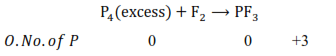However, if $\mathrm{P}_{4}$ is treated with an excess of $\mathrm{F}_{2}$, then $\mathrm{PF}_{5}$ will be produced, wherein the $0 . \mathrm{N}$. of $\mathrm{P}$ is $+5$ in $\mathrm{PF}_{5}$.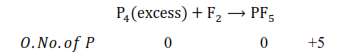(ii) K acts as a reducing agent, whereas $\mathrm{O}_{2}$ is an oxidising agent. If an excess of $\mathrm{K}$ reacts with $\mathrm{O}_{2}$, then $\mathrm{K}_{2} \mathrm{O}$ will be formed, wherein the $0 . \mathrm{N}$. of 0 is $-2 \mathrm{in} \mathrm{K}_{2} \mathrm{O}$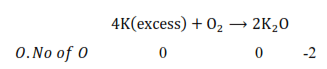However, if $\mathrm{K}$ reacts with an excess of $\mathrm{O}_{2}$, then $\mathrm{K}_{2} \mathrm{O}_{2}$ will be formed, wherein the $0 . \mathrm{N}$. of $\mathrm{O}$ is $-1$ in $\mathrm{K}_{2} \mathrm{O}_{2}$.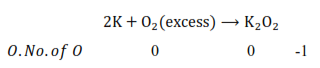On the other hand, if $C$ is burnt in an excess of $0_{2}$, then $\mathrm{CO}_{2}$ will be produced, wherein the 0. N. of $C$ is $+4$.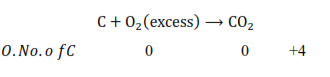Question 12. How do you count for the following observations?

(a) Though alkaline potassium permanganate and acidic potassium permanganate both are used as oxidants, yet in the manufacture of benzoic acid from toluene we use alcoholic potassium permanganate as an oxidant, Why? Write a balanced redox equation for the reaction.

(b) When concentrated sulphuric acid is added to an inorganic mixture containing chloride, we get colourless pungent smelling gas HCl, but if the mixture contains bromide then we get red vapour of bromine. Why? $\rangle$

Solution: (a) In the manufacture of benzoic acid from toluene, alcoholic potassium permanganate is used as an oxidant because of the following reasons.

(i) In a neutral medium, $\mathrm{OH}^{-}$ ions are produced in the reaction itself. As a result, the cost of adding an acid or a base can be reduced.

$\mathrm{MnC}_{3}+2 \mathrm{H}_{2} \mathrm{O}+\mathrm{3e}^{-} \rightarrow \mathrm{MnO}_{2}+4 \mathrm{OH}^{-}$

(ii) $\mathrm{KMnO}_{4}$ and alcohol both are polar and homogeneous to each other Toluene and alcohol are also homogeneous to each other because both are organic compounds. Reactions can proceed at a faster rate in a homogeneous medium than in a heterogeneous medium.

Hence, in alcohol, $\mathrm{KMnO}_{4}$ and toluene can react at a faster rate.

The balanced redox equation for the reaction in a neutral medium is give as below: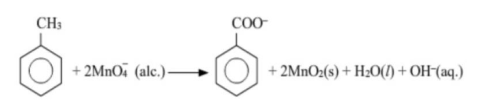(b) When conc. $\mathrm{H}_{2} \mathrm{SO}_{4}$ is added to an inorganic mixture containing bromide, initially $\mathrm{HBr}$ is produced. HBr, being a strong reducing agent reduces $\mathrm{H}_{2} \mathrm{SO}_{4}$ to $\mathrm{SO}_{2}$ with the evolution of red vapour of bromine.

$2 \mathrm{NaBr}+2 \mathrm{H}_{2} \mathrm{SO}_{4} \rightarrow 2 \mathrm{NaHSO}_{4}+2 \mathrm{HBr}$

$2 \mathrm{HBr}+\mathrm{H}_{2} \mathrm{SO}_{4} \rightarrow \mathrm{Br}_{2}+\mathrm{SO}_{2}+2 \mathrm{H}_{2} \mathrm{O}$

But, when conc. $\mathrm{H}_{2} \mathrm{SO}_{4}$ is added to an inorganic mixture containing chloride, a pungent smelling gas (HCl) is evolved. HCl, being a weak reducing agent, cannot reduce $\mathrm{H}_{2} \mathrm{SO}_{4}$ to $\mathrm{SO}_{2}$.

$2 \mathrm{NaCl}+2 \mathrm{H}_{2} \mathrm{SO}_{4} \rightarrow 2 \mathrm{NaHSO}_{4}+2 \mathrm{HCl}$

Question 13. Identify the substance oxidised reduced, oxidising agent and reducing agent for each of the following reactions:

(a) $2 \mathrm{AgBr}(\mathrm{s})+\mathrm{C}_{6} \mathrm{H}_{6} \mathrm{O}_{2}(\mathrm{aq}) \rightarrow 2 \mathrm{Ag}(\mathrm{s})$

$+2 \mathrm{HBr}(\mathrm{aq})+\mathrm{C}_{5} \mathrm{H}_{4} \mathrm{O}_{2}(\mathrm{aq})$

(b)$\mathrm{HCHO}(\mathrm{l})+2\left[\mathrm{Ag}\left(\mathrm{NH}_{3}\right)_{2}\right]^{+}(\mathrm{aq})+3 \mathrm{OH}^{-}$

$(\mathrm{aq}) \rightarrow 2 \mathrm{Ag}(\mathrm{s})+\mathrm{HCOO}^{-}(\mathrm{aq})+$

$4 \mathrm{NH}_{3}(\mathrm{aq})+2 \mathrm{H}_{2} \mathrm{O}(\mathrm{l})$

(c) $\mathrm{HCHO}(\mathrm{l})+2 \mathrm{Cu}^{2+}(\mathrm{aq})+5 \mathrm{OH}^{-}(\mathrm{aq})$

$\rightarrow \mathrm{Cu}_{2} \mathrm{O}(\mathrm{s})+\mathrm{HCOO}^{-}(\mathrm{aq})+$

$3 \mathrm{H}_{2} \mathrm{O}(\mathrm{l})$

(d) $\mathrm{N}_{2} \mathrm{H}_{4}(\mathrm{l})+2 \mathrm{H}_{2} \mathrm{O}_{2}(\mathrm{l}) \rightarrow \mathrm{N}_{2}(\mathrm{~g})+4 \mathrm{H}_{2} \mathrm{O}(\mathrm{l})$

(e) $\mathrm{Pb}(\mathrm{s})+\mathrm{PbO}_{2}(\mathrm{~s})+2 \mathrm{H}_{2} \mathrm{SO}_{4}(\mathrm{aq})$

$\rightarrow 2 \mathrm{PbSO}_{4}(\mathrm{~s})+2 \mathrm{H}_{2} \mathrm{O}(\mathrm{l})$

Solution. (a) $2 \mathrm{AgBr}(\mathrm{s})+\mathrm{C}_{6} \mathrm{H}_{6} \mathrm{O}_{2}(\mathrm{aq})$

$\rightarrow 2 \mathrm{Ag}(\mathrm{s})+2 \mathrm{HBr}(\mathrm{aq})+\mathrm{C}_{6} \mathrm{H}_{4} \mathrm{O}_{2}(\mathrm{aq})$

Reduction : $e^{-}+\mathrm{Ag}^{+} \rightarrow \mathrm{Ag}(\mathrm{s})$

Oxidation : $\mathrm{C}_{6} \mathrm{H}_{6} \mathrm{O}_{2}(\mathrm{aq})$

$\rightarrow \mathrm{C}_{6} \mathrm{H}_{4} \mathrm{O}_{2}(\mathrm{aq})+2 \mathrm{H}^{+}+2 e^{-}$

$\begin{array}{lll}\text { O.No of } \mathrm{C} & 1 / 3 & 0\end{array}$

Oxidised substance $\rightarrow \mathrm{C}_{6} \mathrm{H}_{6} \mathrm{O}_{2}$

Reduced substance $\rightarrow$ AgBr

Oxidising agent $\rightarrow \mathrm{AgBr}$

Reducing agent $\rightarrow \mathrm{C}_{6} \mathrm{H}_{6} \mathrm{O}_{2}$

(b) $\mathrm{HCHO}(\mathrm{l})+2\left[\mathrm{Ag}\left(\mathrm{NH}_{3}\right)_{2}\right]^{+}$

$(\mathrm{ag})+3 \mathrm{OH}^{-}(\mathrm{aq}) \rightarrow 2 \mathrm{Ag}(\mathrm{s})+$

$\mathrm{HCOO}^{-}(\mathrm{aq})+4 \mathrm{NH}_{3}(\mathrm{aq})+2 \mathrm{H}_{2} \mathrm{O}(\mathrm{l})$

Oxidation : $\mathrm{H}_{2} \mathrm{O}(\mathrm{l})+\mathrm{HCHO}(\mathrm{l})$

$\rightarrow \mathrm{HCOO}^{-}(\mathrm{aq})+3 \mathrm{H}^{+}+2 e^{-}$

Reduction : $\left[\mathrm{Ag}\left(\mathrm{NH}_{3}\right)_{2}\right]^{+}(\mathrm{aq})+e^{-}$

$\rightarrow \mathrm{Ag}(\mathrm{s})+2 \mathrm{NH}_{3}(\mathrm{aq})+2 \mathrm{H}_{2} \mathrm{O}(\mathrm{l})$

Oxidised substance $\rightarrow$ HCHO

Reduced substance $\rightarrow\left[\mathrm{Ag}\left(\mathrm{NH}_{3}\right)_{2}\right]^{+}$

Oxidising agent $\rightarrow\left[\mathrm{Ag}\left(\mathrm{NH}_{3}\right)_{2}\right]^{+}$

Reducing agent $\rightarrow$ HCHO

(c) $\quad \mathrm{HCHO}(\mathrm{l})+2 \mathrm{Cu}^{2+}(\mathrm{aq})$

$+5 \mathrm{OH}^{-}(\mathrm{aq}) \rightarrow \mathrm{Cu}_{2} \mathrm{O}(\mathrm{s})+$

$\mathrm{HCOO}^{-}(\mathrm{aq})+3 \mathrm{H}_{2} \mathrm{O}(\mathrm{l})$

Reduction: $\quad e^{-}+\mathrm{Cu}^{2+}(\mathrm{aq}) \rightarrow \mathrm{Cu}^{1+}(\mathrm{aq})$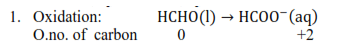Oxidised substance $\rightarrow$ HCHO

Reduced substance $\rightarrow \mathrm{Cu}^{2+}$

Oxidising agent $\rightarrow \mathrm{Cu}^{2+}$

Reducing agent $\rightarrow$ HCHO

(d) $\mathrm{N}_{2} \mathrm{H}_{4}(\mathrm{l})+2 \mathrm{H}_{2} \mathrm{O}_{2}(\mathrm{l})$

$\rightarrow \mathrm{N}_{2}(\mathrm{~g})+4 \mathrm{H}_{2} \mathrm{O}(\mathrm{l})$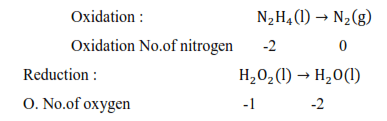Dxidised substance $\rightarrow \mathrm{N}_{2} \mathrm{H}_{4}$

Reduced substance $\rightarrow \mathrm{H}_{2} \mathrm{O}_{2}$

Oxidising agent $\rightarrow \mathrm{H}_{2} \mathrm{O}_{2}$

Reducing agent $\rightarrow \mathrm{N}_{2} \mathrm{H}_{4}$

(e) $\mathrm{Pb}(\mathrm{s})+\mathrm{PbO}_{2}(\mathrm{~s})+2 \mathrm{H}_{2} \mathrm{SO}_{4}(\mathrm{aq})$

$\rightarrow 2 \mathrm{PbSO}_{4}(\mathrm{~s})+2 \mathrm{H}_{2} \mathrm{O}(\mathrm{l})$

Oxidation : $\mathrm{Pb}(\mathrm{s}) \rightarrow \mathrm{Pb}^{+2}(\mathrm{~s})+2 \mathrm{e}^{-}$

Reduction $: 2 \mathrm{e}^{-}+\mathrm{Pb}^{+4}(\mathrm{~s}) \rightarrow \mathrm{Pb}^{+2}(\mathrm{~s})$

Oxidised substance $\rightarrow \mathrm{Pb}$

Reduced substance $\rightarrow \mathrm{PbO}_{2}$

Oxidising agent $\rightarrow \mathrm{PbO}_{2}$

Reducing agent $\rightarrow \mathrm{Pb}$

Question 14. Consider the reactions :

$2 \mathrm{~S}_{2} \mathrm{O}_{3}^{2-}(\mathrm{aq})+\mathrm{I}_{2}(\mathrm{~s})$

$\rightarrow \mathrm{S}_{4} \mathrm{O}_{6}^{2-}(\mathrm{aq})+2 \mathrm{I}^{-}(\mathrm{aq})$

$\mathrm{S}_{2} \mathrm{O}_{3}^{2-}(\mathrm{aq})+2 \mathrm{Br}_{2}(\mathrm{l})+5 \mathrm{H}_{2} \mathrm{O}(\mathrm{l})$

$\rightarrow 2 \mathrm{SO}_{4}^{2-}(\mathrm{aq})+4 \mathrm{Br}^{-}(\mathrm{aq})+10 \mathrm{H}^{+}(\mathrm{aq})$

Why does the same reductant, thiosulphate react differently with iodine and bromine?

Solution: $2 \mathrm{~S}_{2} \mathrm{O}_{3}^{2-}(\mathrm{aq})+\mathrm{I}_{2}(\mathrm{~s})$

$\mathrm{S}_{4} \mathrm{O}_{6}^{2-}(\mathrm{aq})+2 \mathrm{I}^{-}(\mathrm{aq})$

Oxidation : $2 \mathrm{~S}_{2} \mathrm{O}_{3}^{2-}$ (aq)

$\rightarrow \mathrm{S}_{4} 0_{6}^{2-}(\mathrm{aq})+2 \mathrm{e}^{-}$

$\mathrm{S}_{2} \mathrm{O}_{3}^{2-}$ thiosulphate get oxidized so it is reducing agent

$\mathrm{S}_{2} \mathrm{O}_{3}^{2-}(\mathrm{aq})+2 \mathrm{Br}_{2}(\mathrm{l})+5 \mathrm{H}_{2} \mathrm{O}(\mathrm{l})$

$2 \mathrm{SO}_{4}^{2-}(\mathrm{aq})+4 \mathrm{Br}^{-}(\mathrm{aq})+10 \mathrm{H}^{+}(\mathrm{aq})$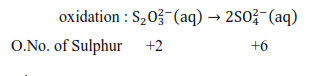reduction : $\mathrm{Br}_{2}(\mathrm{l})+2 \mathrm{e}^{-} \rightarrow 2 \mathrm{Br}^{-}(\mathrm{aq})$

$\mathrm{S}_{2} \mathrm{O}_{3}^{2-}$ get oxidized so it is reducing agent while $\mathrm{Br}_{2}$ get reduced to it oxidizing agent here.

Question 15. Justify giving reactions that among halogens, fluorine is the best oxidant and among hydrohalic compounds, hydroiodic acid is the best reductant.

Solution: $\mathrm{F}_{2}$ can oxidize $\mathrm{C}$ - to $\mathrm{Cl}_{2}, \mathrm{Br}^{-}$ to $\mathrm{Br}_{2}$, and $\mathrm{I}^{-}$ to $\mathrm{I}_{2}$

$\mathrm{F}_{2}+2 \mathrm{Cl}^{-} \rightarrow 2 \mathrm{~F}^{-1}+\mathrm{Cl}_{2}$

$\mathrm{F}_{2}+2 \mathrm{Br}^{-} \rightarrow \mathrm{Br}_{2}+2 F^{-1}$

$\mathrm{F}_{2}+2 \mathrm{I}^{-} \rightarrow \mathrm{I}_{2}+2 F^{-1}$

On the other hand, $\mathrm{Cl}_{2}, \mathrm{Br}_{2}$, and $\mathrm{I}_{2}$ cannot oxidize $\mathrm{F}^{-}$ to $\mathrm{F}_{2}$. The oxidizing power of halogens

increases in the order of $\mathrm{I}_{2}<\mathrm{Br}_{2}<\mathrm{Cl}_{2}<\mathrm{F}_{2}$. Hence, fluorine is the best oxidant among halogens. Standard Reduction Potential value is highest for $\mathrm{F}_{2}$

$2 \mathrm{HI}+\mathrm{H}_{2} \mathrm{SO}_{4} \rightarrow \mathrm{I}_{2}+\mathrm{SO}_{2}+2 \mathrm{H}_{2} \mathrm{O}$

$2 \mathrm{HBr}+\mathrm{H}_{2} \mathrm{SO}_{4} \rightarrow \mathrm{Br}_{2}+\mathrm{SO}_{2}+2 \mathrm{H}_{2} \mathrm{O}$

$\mathrm{I}^{-}$ can reduce $\mathrm{Cu}^{2+}$ to $\mathrm{Cu}^{+}$

$4 \mathrm{I}^{-}(\mathrm{aq})+2 \mathrm{Cu}^{2+}(\mathrm{aq})$’

$\rightarrow \mathrm{Cu}_{2} \mathrm{I}_{2}(\mathrm{~s})+\mathrm{I}_{2}(\mathrm{aq})$

Hence, hydroiodic acid is the best reductant among hydrohalic compounds.

Thus, the reducing power of hydrohalic acids increases in the order of $\mathrm{HF}<\mathrm{HCl}<$ $\mathrm{HBr}<\mathrm{HI}$. Due to top to bottom reducing character increases as to bond length increase.

Question 16. Why does the following reaction occur?

$\mathrm{Xe} \mathrm{O}_{6}^{4-}(\mathrm{aq})+2 \mathrm{~F}^{-}(\mathrm{aq})+6 \mathrm{H}^{+}(\mathrm{aq})$

$\rightarrow \mathrm{XeO}_{3}(\mathrm{~g})+\mathrm{F}_{2}(\mathrm{~g})+3 \mathrm{H}_{2} \mathrm{O}(\mathrm{l})$

What conclusion about the compound $\mathrm{Na}_{4} \mathrm{XeO}_{6}$ (of which $\mathrm{XeO}_{6}^{4-}$ is a part) can be drawn from the reaction.

Solution: The given reaction occurs because $\mathrm{XeO}_{6}^{4-}$ oxidises $\mathrm{F}^{-}$ and $\mathrm{F}^{-}$ reduces $\mathrm{XeO}_{6}^{4-}$

$\mathrm{XeO}_{6}^{4-}(\mathrm{aq})+2 \mathrm{~F}^{-}(\mathrm{aq})+6 \mathrm{H}^{+}(\mathrm{aq})$

$\rightarrow \mathrm{XeO}_{3}(\mathrm{~g})+\mathrm{F}_{2}(\mathrm{~g})+3 \mathrm{H}_{2} \mathrm{O}(\mathrm{l})$

In this reaction, the O.N. (0.N.) of Xe decreases from $+8$ in $\mathrm{XeO}_{6}^{4-}$ to $+6$ in $\mathrm{XeO}_{3}$ and the $0 . \mathrm{N}$. of $\mathrm{F}$ increases from $-1$ in $\mathrm{F}^{-}$ to zero in $\mathrm{F}_{2}$ Hence, we can

conclude that $\mathrm{Na}_{4} \mathrm{Xe} 0_{6}$ is a stronger oxidising agent than $\mathrm{F}^{-}$.

Question 17. Consider the reactions:

(A) $\mathrm{H}_{3} \mathrm{PO}_{2}$ (aq) $+4 \mathrm{AgNO}_{3}(\mathrm{aq})+2 \mathrm{H}_{2} \mathrm{O}(\mathrm{l})$

$\rightarrow \mathrm{H}_{3} \mathrm{PO}_{4}(\mathrm{aq})+4 \mathrm{Ag}(\mathrm{s})+$$4 \mathrm{HNO}_{3}(\mathrm{aq}) (B) \mathrm{H}_{3} \mathrm{PO}_{2}(\mathrm{aq})+2 \mathrm{CuSC}_{4}(\mathrm{aq})+2 \mathrm{H}_{2} \mathrm{O}(\mathrm{l}) \rightarrow \mathrm{H}_{3} \mathrm{PO}_{4}(\mathrm{aq})+2 \mathrm{Cu}(\mathrm{s})+$$\mathrm{H}_{2} \mathrm{SO}_{4}(\mathrm{aq})$

(C) $\quad \mathrm{C}_{6} \mathrm{H}_{5} \mathrm{CHO}(\mathrm{l})+2\left[\mathrm{Ag}\left(\mathrm{NH}_{3}\right)_{2}\right]^{+}(\mathrm{aq})+3 \mathrm{OH}^{-}(\mathrm{aq})$

$\rightarrow \mathrm{C}_{6} \mathrm{H}_{5} \mathrm{COO}^{-}(\mathrm{aq})+$

$2 \mathrm{Ag}(\mathrm{s})+4 \mathrm{NH}_{3}(\mathrm{aq})+2 \mathrm{H}_{2} \mathrm{O}(\mathrm{l})$

(D) $\mathrm{C}_{6} \mathrm{H}_{5} \mathrm{CHO}(\mathrm{l})+2 \mathrm{Cu}^{2+}(\mathrm{aq})$

$+50 \mathrm{H}^{-}(\mathrm{aq}) \rightarrow$ No change observed.

What inference do you draw about the behaviour of $\mathrm{Ag}^{+}$ and $\mathrm{Cu}^{2+}$ from these reactions?

Solution: (A) $\mathrm{Ag}^{+}(\mathrm{aq})+\mathrm{e}^{-} \rightarrow \mathrm{Ag}(\mathrm{s})$v

(B) $\mathrm{Cu}^{+2}(\mathrm{aq})+2 \mathrm{e}^{-} \rightarrow \mathrm{Cu}(\mathrm{s})$

(C) $\left[\mathrm{Ag}\left(\mathrm{NH}_{3}\right)_{2}\right]^{+}(\mathrm{aq})+\mathrm{e}^{-} \rightarrow \mathrm{Ag}(\mathrm{s})$

$A g^{+}$ and $\mathrm{Cu}^{2+}$ act as oxidising agents in reactions (a) and (b)respectively.

In reaction (c), $A g^{+}$ oxidises $\mathrm{C}_{6} \mathrm{H}_{5} \mathrm{CHO}$ to $\mathrm{C}_{6} \mathrm{H}_{5} \mathrm{COO}^{-}$, but in reaction (d), $\mathrm{Cu}^{2+}$

cannot oxidise $\mathrm{C}_{6} \mathrm{H}_{5} \mathrm{CH} 0$.

Hence, $\mathrm{Cu}^{2+}$ is a stronger oxidising agent than $\mathrm{Cu}^{2+}$.

Question 18. Balance the following redox reactions by ion - electron method :

(A) $\quad \mathrm{MnO}_{4}^{-}(\mathrm{aq})+\mathrm{I}^{-}(\mathrm{aq})$

$\mathrm{MnO}_{2}(\mathrm{~s})+\mathrm{I}_{2}(\mathrm{~s})$ (in basic medium)

(B) $\quad \mathrm{MnO}_{4}^{-}(\mathrm{aq})+\mathrm{SO}_{2}(\mathrm{~g})$

$\rightarrow \mathrm{Mn}^{2+}(\mathrm{aq})+\mathrm{HSO}_{4}^{-}(\mathrm{aq})$ (in acidic solution)

(C) $\mathrm{H}_{2} \mathrm{O}_{2}(\mathrm{aq})+\mathrm{Fe}^{2+}(\mathrm{aq})$

$\rightarrow \mathrm{Fe}^{3+}(\mathrm{aq})+\mathrm{II}_{2} \mathrm{O}(\mathrm{l})$ (in aeidie solution)

(D) $\quad \mathrm{Cr}_{2} \mathrm{O}_{7}^{2-}+\mathrm{SO}_{2}(\mathrm{~g}) \rightarrow \mathrm{Cr}^{3+}(\mathrm{aq})$

$+\mathrm{SO}_{4}^{2-}(\mathrm{aq})$ (in acidic solution)

Solution: (a) Step 1: The two half reactions involved in the given reaction are:

Oxidation half reaction: $\mathrm{I}^{-1}(\mathrm{aq}) \rightarrow \mathrm{I}_{2}(\mathrm{~s})$

Reduction half reaction: $M \mathrm{nO}_{4}^{-}(\mathrm{aq}) \rightarrow \mathrm{M} \mathrm{nO}_{2}(\mathrm{aq})$

Step 2: Balancing I in the oxidation half reaction, we have:

Now, to balance the atom, we multiply $\mathrm{I}^{-}$ with 2 into the LHS of the reaction

$2 \mathrm{I}^{-}(\mathrm{aq}) \rightarrow \mathrm{I}_{2}(\mathrm{~s})$

Now, to balance the charge, we add $2 \mathrm{e}^{-}$ to the RHS of the reaction.

$2 \mathrm{I}^{-}(\mathrm{aq}) \rightarrow \mathrm{I}_{2}(\mathrm{~s})+2 \mathrm{e}^{-}$

Step 3: In the reduction half-reaction, Balance the atoms other than $\mathrm{O}$ and

H in each half-reaction individually. Here the to balance the atoms add $\mathrm{H}_{2} \mathrm{O}$ to balance $\mathrm{O}$ atoms and $\mathrm{H}^{+}$

to balance $\mathrm{H}$ atoms.

Thus,we get $: \mathrm{MnO}_{4}^{-}(\mathrm{aq})+4 \mathrm{H}^{+}$

$\rightarrow \mathrm{MnO}_{2}(\mathrm{aq})+2 \mathrm{H}_{2} \mathrm{O}$

Step 4: The reaction occurs in a basic medium, so we add $40 \mathrm{H}^{-}$ ions to the RHS and right-hand side.

$\mathrm{MnO}_{4}^{-}(\mathrm{aq})+4 \mathrm{H}^{+}+4 \mathrm{OH}^{-}$

$\rightarrow \mathrm{MnO}_{2}(\mathrm{aq})+2 \mathrm{H}_{2} \mathrm{O}+4 \mathrm{OH}^{-}$

$=\mathrm{MnO}_{4}^{-}(\mathrm{aq})+2 \mathrm{H}_{2} \mathrm{O}$

$\rightarrow \mathrm{MnC}_{2}(\mathrm{aq})+4 \mathrm{OH}^{-}$

Step $5:$ To balance the charge add 3 electrons are added to the LHS of the reaction.

$3 \mathrm{e}^{-}+\mathrm{MnO}_{4}^{-}(\mathrm{aq})+2 \mathrm{H}_{2} \mathrm{O} \rightarrow \mathrm{MnO}_{2}(\mathrm{aq})+4 \mathrm{OH}^{-}$

Step 6: Equalising the number of electrons by multiplying the oxidation half-reaction by 3 and the reduction half-reaction by 2 , we have:

$6 \mathrm{I}^{-}(\mathrm{aq}) \rightarrow 3 \mathrm{I}_{2}(\mathrm{~s})+6 \mathrm{e}^{-}$

$2 \mathrm{MnO}_{4}^{-}(\mathrm{cq})+4 \mathrm{H}_{2} \mathrm{O}+6 \mathrm{e}^{-}$

$\rightarrow \mathrm{MnO}_{2}(\mathrm{~s})+8 \mathrm{OH}^{-}(\mathrm{aq})$

Step 7: Adding the two half-reactions, we have the net balanced redox reaction as:

$6 \mathrm{I}^{-}(\mathrm{aq})+2 \mathrm{MnO}_{4}^{-}(\mathrm{aq})+4 \mathrm{H}_{2} \mathrm{O}(\mathrm{l})$

$\rightarrow 3 \mathrm{I}_{2}(\mathrm{~s})+2 \mathrm{MnO}_{2}(\mathrm{~s})+8 \mathrm{OH}^{-}(\mathrm{aq})$

(b) $\quad \mathrm{MnO}_{4}^{-}(\mathrm{aq})+\mathrm{SO}_{2}(\mathrm{~g}) \rightarrow \mathrm{Mn}^{2+}(\mathrm{aq})+\mathrm{HSO}_{4}^{-}(\mathrm{aq})(\mathrm{in}$

acidic solution)Following the steps as in part (a), we have the oxidation half-reaction as:

$\mathrm{SO}_{2}(\mathrm{~g}) \rightarrow \mathrm{HSO}_{4}^{-}(\mathrm{aq})$

Step 1: In the reduction half-reaction, Balance the atoms other than $\mathrm{O}$ and

H in each half-reaction individually. Here the to balance the atoms add $\mathrm{H}_{2} \mathrm{O}$ to balance $\mathrm{O}$ atoms and $H^{+}$ to balance $\mathrm{H}$ atoms.

$2 \mathrm{H}_{2} \mathrm{O}(\mathrm{l})+\mathrm{SO}_{2}(\mathrm{~g}) \rightarrow \mathrm{HSO}_{4}^{-}(\mathrm{aq})+3 \mathrm{H}^{+}$

Step 2:To balance the charge add 2 electrons are added to the RHS of the reaction.

$2 \mathrm{H}_{2} \mathrm{O}(\mathrm{l})+\mathrm{SO}_{2}(\mathrm{~g}) \rightarrow \mathrm{HSO}_{4}^{-}(\mathrm{aq})+3 \mathrm{H}^{+}+2 \mathrm{e}^{-}$

the reduction half reaction as:

Step 3: In the reduction half-reaction, Balance the atoms other than $\mathrm{O}$ and

$\mathrm{H}$ in each half-reaction individually. Here the to balance the atoms add $\mathrm{H}_{2} \mathrm{O}$ to balance $\mathrm{O}$ atoms and $H^{+}$ to balance $\mathrm{H}$ atoms.

$\mathrm{MnO}_{4}^{-}(\mathrm{aq})+8 \mathrm{H}^{+}(\mathrm{aq}) \rightarrow \mathrm{Mn}^{2+}(\mathrm{aq})+4 \mathrm{H}_{2} \mathrm{O}(\mathrm{l})$

Step $4:$ To balance the charge add 5 electrons are added to the LHS of the reaction.

$\mathrm{MnO}_{4}^{-}(\mathrm{aq})+8 \mathrm{H}^{+}(\mathrm{aq})+5 \mathrm{e}^{-}$

$\rightarrow \mathrm{Mn}^{2+}(\mathrm{aq})+4 \mathrm{H}_{2} \mathrm{O}(\mathrm{l})$

Step 5 :Multiplying the oxidation half reaction by 5 and the reduction half reaction by 2, and then by adding them, we have the net balanced redox reaction as:

$\left.2 \mathrm{MnO}_{4}^{-1} \mathrm{aq}\right)+5 \mathrm{SC}_{2}(\mathrm{~g})+2 \mathrm{H}_{2} \mathrm{O}(\mathrm{l})+\mathrm{H}^{+}(\mathrm{aq})$

$\rightarrow 2 \mathrm{Mn}^{2+}(\mathrm{aq})+5 \mathrm{HSO}_{4}^{-}(\mathrm{aq})$

(c) Following the steps as in part (a), we have the oxidation half reaction as:

Step 1: To balance the charge add 1 electron in RHS

$\mathrm{Fe}^{2+}(\mathrm{aq}) \rightarrow \mathrm{Fe}^{3+}(\mathrm{aq})+\mathrm{e}^{-}$

And the reduction half reaction as:

Step 2: Balance the atoms other than $\mathrm{O}$ and $\mathrm{H}$ in each half-reaction individually. Here the to balance the atoms add $H_{2} O$ to balance $O$ atoms and $H^{+}$ to balance $\mathrm{H}$ atoms.

$\mathrm{H}_{2} \mathrm{O}_{2}(\mathrm{aq})+2 \mathrm{H}^{+}(\mathrm{aq}) \rightarrow 2 \mathrm{H}_{2} \mathrm{O}(\mathrm{l})$

Step 3:To balance the charge add 2 electrons are added to the LHS of the reaction.

$2 e^{-}+\mathrm{H}_{2} \mathrm{O}_{2}(\mathrm{aq})+2 \mathrm{H}^{+}(\mathrm{aq}) \rightarrow 2 \mathrm{H}_{2} \mathrm{O}(\mathrm{l})$

Step 4: Multiplying the oxidation half reaction by 2 and then adding it to the reduction half reaction, we have the net balanced redox reaction as:

$\mathrm{H}_{2} \mathrm{O}_{2}(\mathrm{aq})+2 \mathrm{Fe}^{2+}(\mathrm{aq})+2 \mathrm{H}^{+}(\mathrm{aq})$

$\rightarrow 2 \mathrm{Fe}^{3+}(\mathrm{aq})+2 \mathrm{H}_{2} \mathrm{O}(\mathrm{l})$

(d) Following the steps as in part (a), we have the oxidation half reaction as:

Step 1: Balance the atoms other than $\mathrm{O}$ and $\mathrm{H}$ in each half-reaction individually. Here the to balance the atoms add $\mathrm{H}_{2} \mathrm{O}$ to balance $\mathrm{O}$ atoms and $H^{+}$ to balance $\mathrm{H}$ atoms.

$\mathrm{SO}_{2}(\mathrm{~g})+2 \mathrm{H}_{2} \mathrm{O}(\mathrm{l}) \rightarrow \mathrm{SO}_{4}^{2-}+4 \mathrm{H}^{+}(\mathrm{aq})$

Step 2: To balance the charge add 2 electrons are added to the RHS of the reaction.

$\mathrm{SO}_{2}(\mathrm{~g})+2 \mathrm{H}_{2} \mathrm{O}(\mathrm{l}) \rightarrow \mathrm{SO}_{4}^{2-}+4 \mathrm{H}^{+}(\mathrm{aq})+2 \mathrm{e}^{-}$

the reduction half reaction as:

Step 3: Balance the $\mathrm{Cr}$ atom multiply the $\mathrm{Cr}^{3+}$ with 2 in RHS

$\mathrm{Cr}_{2} \mathrm{O}_{7}^{2-} \rightarrow 2 \mathrm{Cr}^{3+}(\mathrm{aq})$

Step 4: Balance the atoms other than $\mathrm{O}$ and $\mathrm{H}$ in each half-reaction individually. Here the to balance the atoms add $\mathrm{H}_{2} \mathrm{O}$ to balance $\mathrm{O}$ atoms and $H^{+}$ to balance $\mathrm{H}$ atoms.

$\mathrm{Cr}_{2} \mathrm{O}_{7}^{2-}+14 \mathrm{H}^{+}(\mathrm{aq})$

$\rightarrow 2 \mathrm{Cr}^{3+}(\mathrm{aq})+7 \mathrm{H}_{2} \mathrm{O}(\mathrm{l})$

Step 5: To balance the charge add 6 electrons are added to the LHS of the reaction.

$\mathrm{Cr}_{2} \mathrm{O}_{7}^{2-}+14 \mathrm{H}^{+}(\mathrm{aq})+6 \mathrm{e}^{-}$

$\rightarrow 2 \mathrm{Cr}^{3+}(\mathrm{aq})+7 \mathrm{H}_{2} \mathrm{O}(\mathrm{l})$

Multiplying the oxidation half reaction by 3 and then adding it to the reduction half reaction, we have the net balanced redox reaction as:

$\mathrm{Cr}_{2} \mathrm{O}_{7}^{2-}+3 \mathrm{SO}_{2}(\mathrm{~g})+2 \mathrm{H}^{+}(\mathrm{a} \mathrm{p})$

$\rightarrow 2 \mathrm{C}^{3+}(\mathrm{aq})+3 \mathrm{SO}_{4}^{2-}+\mathrm{H}_{2} \mathrm{O}(\mathrm{l})$

Question 19. Balance the following equations I n basic medium by ion-electron method and O.N. methods and identify the oxidising agent and the reducing agent.

$\mathrm{P}_{4}(\mathrm{~s})+\mathrm{OH}^{-}(\mathrm{aq}) \rightarrow \mathrm{PH}_{3}(\mathrm{~g})+\mathrm{HPO}_{2}^{-}(\mathrm{aq})$

$\mathrm{N}_{2} \mathrm{H}_{4}(\mathrm{l})+\mathrm{ClO}_{3}^{-}(\mathrm{aq}) \rightarrow \mathrm{NO}(\mathrm{G})+\mathrm{Cl}^{-}(\mathrm{G})$

$\mathrm{Cl}_{2} \mathrm{O}_{7}(\mathrm{~g})+\mathrm{H}_{2} \mathrm{O}_{2}(\mathrm{aq})$

$\rightarrow \mathrm{ClC}_{2}(\mathrm{aq})+\mathrm{O}_{2}(\mathrm{~g})+\mathrm{H}^{+}$

Solution: (a)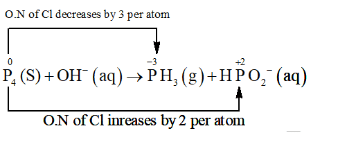The O. N. (O.N.) of $P$ decreases from 0 in $P_{4}$ to $-3$ in $P H_{3}$ and increases from 0 in $\mathrm{P}_{4}$ to $+2$ in $\mathrm{HPO}_{2}^{-}$. Hence, $\mathrm{P}_{4}$ acts both as an oxidizing agent and a reducing agent in this reaction.

Ion-electron method:

The oxidation half equation is:

$\mathrm{P}_{4}(\mathrm{~s}) \rightarrow \mathrm{HPO}_{2}^{-}(\mathrm{aq})$

The $\mathrm{P}$ atom is balanced as:

$\mathrm{P}_{4} \rightarrow 4 \mathrm{HPO}_{2}^{-}(\mathrm{aq})$

The 0 atom is balanced by adding $8 \mathrm{H}_{2} 0$ molecules:

$\mathrm{P}_{4}+8 \mathrm{H}_{2} \mathrm{O} \rightarrow 4 \mathrm{HPO}_{2}^{-}(\mathrm{aq})$

The $\mathrm{H}$ atom is balanced by adding $12 \mathrm{H}^{+}$ ions:

$\mathrm{P}_{4}+8 \mathrm{H}_{2} \mathrm{O} \rightarrow 4 \mathrm{HPO}_{2}^{-}(\mathrm{aq})+12 \mathrm{H}^{+}$

The charge is balanced by adding $8 \mathrm{e}^{-}$ as:

$\mathrm{P}_{4}+8 \mathrm{H}_{2} \mathrm{O} \rightarrow 4 \mathrm{HPO}_{2}^{-}(\mathrm{aq})$

$+12 \mathrm{H}^{+}+8 \mathrm{e}^{-} \ldots(\mathrm{i})$

The reduction half equation is:

$\mathrm{P}_{4}(\mathrm{~s}) \rightarrow \mathrm{PH}_{3}(\mathrm{~g})$

The $\mathrm{P}$ atom is balanced as:

$\mathrm{P}_{4} \rightarrow 4 \mathrm{PH}_{3}(\mathrm{~g})$

The $\mathrm{H}$ is balanced by adding $12 \mathrm{H}^{+}$ as:

$\mathrm{P}_{4}+12 \mathrm{H}^{+} \rightarrow 4 \mathrm{PH}_{3}(\mathrm{~g})$

The charge is balanced by adding $12 \mathrm{e}^{-}$ as:

$\mathrm{P}_{4}+12 \mathrm{H}^{+}+12 \mathrm{e}^{-} \rightarrow 4 \mathrm{PH}_{3}(\mathrm{~g}) \ldots$ (ii)

By multiplying equation (i) with 3 and (ii) with 2 and then adding them, the balanced chemical equation can be obtained as:

$\mathrm{HH}_{2}(\mathrm{~s})+24 \mathrm{H}_{2} \mathrm{O} \rightarrow 12 \mathrm{HPO}_{2}^{-}$

$+8 \mathrm{PH}_{3}(\mathrm{~g})+12 \mathrm{H}^{+}$

As, the medium is basic, add $120 \mathrm{H}^{-}$ both sides as:

$5 \mathrm{P}_{4}(\mathrm{~s})+12 \mathrm{H}_{2} \mathrm{O}+12 \mathrm{OH}^{-}$

$12 \mathrm{HPO}_{2}^{-}+8 \mathrm{PH}_{3}(\mathrm{~g})$

This is the balanced equation by ion-electron method

O.N. method:

Let, total no of $\mathrm{P}$ reduced $=\mathrm{x}$

$\therefore$ Total no of $P$ oxidised $=4-x$

$\mathrm{P}_{4}(\mathrm{~s})+\mathrm{OH}^{-} \rightarrow \mathrm{xPH}_{3}(\mathrm{~g})$

$+(4-x) H P O_{2}^{-} \ldots$ (i)

Total decrease in O.N. of $P=x \times 3=3 x$

Total increase in O.N. of $P=(4-x) \times 2=8-2 x$

$\because 3 \mathrm{x}=82 \mathrm{x} \mathrm{x}=8 / 5$ from (i),

$5 \mathrm{P}_{4}(\mathrm{~s})+5 \mathrm{OH}^{-} \rightarrow 8 \mathrm{PH}_{3}(\mathrm{~g})+12 \mathrm{HPO}_{2}^{-}$

$5 \mathrm{P}_{4}(\mathrm{~s})+50 \mathrm{H}^{-} \rightarrow 8 \mathrm{PH}_{3}(\mathrm{~g})+12 \mathrm{HPO}_{2}^{-}$

Since, reaction occurs in basic medium, the charge is balanced by adding $70 \mathrm{H}^{-}$ on LHS as:

$5 \mathrm{P}_{4}(\mathrm{~s})+12 \mathrm{OH}^{-} \rightarrow 8 \mathrm{PH}_{3}(\mathrm{~g})+12 \mathrm{HPO}_{2}^{-}$

The 0 atoms are balanced by adding $12 \mathrm{H}_{2} \mathrm{O}$ as:

$5 \mathrm{P}_{4}(\mathrm{~s})+12 \mathrm{H}_{2} \mathrm{O}+12 \mathrm{OH}^{-}$

$\rightarrow+12 \mathrm{HPO}_{2}^{-}+8 \mathrm{PH}_{3}(\mathrm{~g})$

This is the required balanced equation.

(b)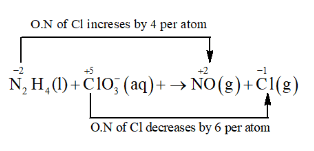The O.N. of $\mathrm{N}$ increases from $-2$ in $\mathrm{N}_{2} \mathrm{H}_{4}$ to $+2$ in NO and the O.N. of $\mathrm{Cl}$ decreases from $+5$ in $\mathrm{ClO}_{3}^{-}$ to $-1$ in $\mathrm{Cl}^{-}$. Hence, in this reaction, $\mathrm{N}_{2} \mathrm{H}_{4}$ is the reducing agent and $\mathrm{ClO}_{3}^{-}$ is the oxidizing agent.

Ion-electron method:

The oxidation half equation is:

$\mathrm{N}_{2}^{-2} \mathrm{H}_{4}(\mathrm{l}) \rightarrow \stackrel{+2}{\mathrm{~N}} \mathrm{O}(\mathrm{g})$

The $\mathrm{N}$ atoms are balanced as:

$\mathrm{N}_{2} \mathrm{H}_{4}(\mathrm{l}) \rightarrow 2 \mathrm{NO}(\mathrm{g})$

The O.N. is balanced by adding 8 electrons as

$\mathrm{N}_{2} \mathrm{H}_{4}(\mathrm{l}) \rightarrow 2 \mathrm{NO}(\mathrm{g})+8 \mathrm{e}^{-}$

The charge is balanced by adding $80 \mathrm{H}^{-}$ ions as:

$\mathrm{N}_{2} \mathrm{H}_{4}(\mathrm{l})+80 \mathrm{H}^{-}(\mathrm{aq}) \rightarrow 2 \mathrm{NO}(\mathrm{g})+8 \mathrm{e}^{-}$

The 0 atoms are balanced by adding $6 \mathrm{H}_{2} \mathrm{O}$ as:

$\mathrm{N}_{2} \mathrm{H}_{4}(\mathrm{l})+8 \mathrm{OH}^{-}(\mathrm{aq})$

$\rightarrow 2 \mathrm{NO}(\mathrm{g})+6 \mathrm{H}_{2} \mathrm{O}(\mathrm{l})+8 \mathrm{e}^{-} \ldots(\mathrm{i})$

The reduction half equation is:

$\stackrel{+2}{\mathrm{C}} 1 \mathrm{O}_{2}^{-}(\mathrm{aq}) \rightarrow \stackrel{+1}{\mathrm{Cl}}^{-}$ (aq)

The O.N. is balanced by adding 6 electrons as:

$\mathrm{Cic}_{3}(\mathrm{aq})+6 \mathrm{e}^{-} \rightarrow \mathrm{Cl}^{-}(\mathrm{aq})$

Th charge is balanced by adding $60 \mathrm{H}^{-}$ ions as:

$\mathrm{ClO}_{3}^{-}(\mathrm{aq})+6 \mathrm{e}^{-} \rightarrow \mathrm{Cl}^{-}(\mathrm{aq})$

$+60 \mathrm{H}^{-}(\mathrm{aq}) \ldots$ (ii)

The balanced equation can be obtained by multiplying equation (i) with 3 and equation (ii) with 4 and then adding them as:

$3 \mathrm{~N}_{2} \mathrm{H}_{4}(1)+4 \mathrm{ClO}_{3}^{-}(\mathrm{aq}) \rightarrow 6 \mathrm{NO}(\mathrm{g})$

$+4 \mathrm{Cl}^{-}(\mathrm{aq})+6 \mathrm{H}_{2} \mathrm{O}(\mathrm{l})$

O.N. method:

Total decrease in O.N. of $\mathrm{N}=2 \times 4=8$

Total increase in O.N. of $\mathrm{Cl}=1 \times 6=6$

On multiplying $\mathrm{N}_{2} \mathrm{H}_{1}$ with 3 and $\mathrm{ClO}_{3}^{-}$ with 4 to balance the increase and decrease in $0 . \mathrm{N} .$, we get:

$3 \mathrm{~N}_{2} \mathrm{H}_{4}(\mathrm{l})+4 \mathrm{ClO}_{3}^{-}(\mathrm{aq}) \rightarrow \mathrm{NO}(\mathrm{g})+\mathrm{Cl}^{-}(\mathrm{aq})$

The $\mathrm{N}$ and $\mathrm{Cl}$ atoms are balanced as:

$3 \mathrm{~N}_{2} \mathrm{H}_{4}(\mathrm{l})+4 \mathrm{ClO}_{3}(\mathrm{aq}) \rightarrow 6 \mathrm{NO}(\mathrm{g})+4 \mathrm{Cl}^{-}(\mathrm{aq})$

The 0 atoms are balanced by adding $6 \mathrm{H}_{2} \mathrm{O}$ as:

$3 \mathrm{~N}_{2} \mathrm{H}_{4}(\mathrm{l})+4 \mathrm{ClO}_{3}^{-}(\mathrm{aq}) \rightarrow 6 \mathrm{NO}(\mathrm{g})$

$+4 \mathrm{Cl}^{-}(\mathrm{aq})+6 \mathrm{H}_{2} \mathrm{O}(\mathrm{l})$

This is the required balanced equation.

(c)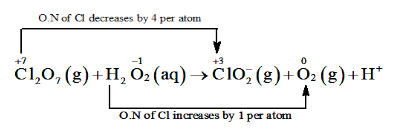The O.N. of $\mathrm{Cl}$ decreases from $+7$ in $\mathrm{Cl}_{2} \mathrm{O}_{7}$ to $+3$ in $\mathrm{ClO}_{2}^{-}$ and the oxidation

number of 0 increases from - 1 in $\mathrm{H}_{2} \mathrm{O}_{2}$ to zero in $\mathrm{O}_{2} .$ Hence, in this reaction, $\mathrm{Cl}_{2} \mathrm{O}_{7}$ is the

oxidizing agent and $\mathrm{H}_{2} \mathrm{O}_{2}$ is the reducing agent.

Ion-electron method:

The oxidation half equation is: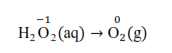The O.N. is balanced by adding 2 electrons as:

$\mathrm{H}_{2} \mathrm{O}_{2}(\mathrm{aq}) \rightarrow \mathrm{O}_{2}(\mathrm{~g})+2 \mathrm{e}^{-}$

The charge is balanced by adding $20 \mathrm{H}^{-}$ ions as:

$\mathrm{H}_{2} \mathrm{O}_{2}(\mathrm{aq}) \rightarrow 2 \mathrm{OH}^{-}(\mathrm{aq})+2 \mathrm{e}^{-}$

The oxygen atoms are balanced by adding $2 \mathrm{H}_{2} \mathrm{O}$ as:

$\mathrm{H}_{2} \mathrm{O}_{2}(\mathrm{aq})+2 \mathrm{OH}^{-}(\mathrm{aq}) \rightarrow+2 \mathrm{H}_{2} \mathrm{O}(\mathrm{l})+2 \mathrm{e}^{-} \ldots(\mathrm{i})$

The reduction half equation is: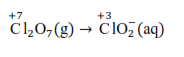The Cl atoms are balanced as:

$\mathrm{Cl}_{2} \mathrm{O}_{7}(\mathrm{~g}) \rightarrow 2 \mathrm{ClO}_{2}^{-}(\mathrm{aq})$

The O.N. is balanced by adding 8 electrons as:

$\mathrm{Cl}_{2} \mathrm{O}_{7}(\mathrm{~g})+8 \mathrm{e}^{-} \rightarrow 2 \mathrm{ClO}_{2}^{-}(\mathrm{aq})$

The charge is balanced by adding $60 \mathrm{H}^{-}$ as:

$\mathrm{Cl}_{2} \mathrm{O}_{7}(\mathrm{~g})+8 \mathrm{c}^{-} \rightarrow 2 \mathrm{ClO}_{2}^{-}(\mathrm{aq})+6 \mathrm{OH}^{-}(\mathrm{aq})$

The oxidation atoms are balanced by adding $3 \mathrm{H}_{2} \mathrm{O}$ as:

$\mathrm{Cl}_{2} \mathrm{O}_{7}(\mathrm{~g})+3 \mathrm{H}_{2} \mathrm{O}(\mathrm{l}) 8 \mathrm{e}^{-}$

$\rightarrow 2 \mathrm{ClC}_{2}(\mathrm{aq})+60 \mathrm{H}^{-}(\mathrm{aq}) \ldots(\mathrm{ii})$

The balanced equation can be obtained by multiplying equation (i) with 4 and adding equation (ii) to it as:

$\mathrm{Cl}_{2} \mathrm{O}_{7}(\mathrm{~g})+4 \mathrm{H}_{2} \mathrm{O}_{2}(\mathrm{aq})+2 \mathrm{OH}^{-}(\mathrm{aq})$

$\rightarrow 2 \mathrm{ClO}_{2}^{-}+4 \mathrm{C}_{2}(\mathrm{~g})+5 \mathrm{H}_{2} \mathrm{O}(\mathrm{l})$

O.N. method:

Total decrease in O.N. of $\mathrm{Cl}_{2} \mathrm{O}_{7}=4 \times 2=8$

Total increase in O.N. of $\mathrm{H}_{2} \mathrm{O}_{2}=2 \times 1=2$

By multiplying $\mathrm{H}_{2} \mathrm{O}_{2}$ and $\mathrm{O}_{2}$ with 4 to balance the increase and decrease in the

O.N., we get:

$\mathrm{Cl}_{2} \mathrm{O}_{7}(\mathrm{~g})+4 \mathrm{H}_{2} \mathrm{O}_{2}(\mathrm{aq})$

$\rightarrow \mathrm{ClO}_{2}^{-}(\mathrm{aq})+4 \mathrm{O}_{2}(\mathrm{~g})$

The Cl atoms are balanced as:

$\mathrm{Cl}_{2} \mathrm{~L}_{7}(\mathrm{~g})+4 \mathrm{H}_{2} \mathrm{O}_{2}(\mathrm{aq})$

$\rightarrow 2 \mathrm{ClO}_{2}^{-}(\mathrm{aq})+40_{2}(\mathrm{~g})$

The 0 atoms are balance $\alpha$ by adding $3 \mathrm{H}_{2} \mathrm{O}$ as:

$\mathrm{Cl}_{2} \mathrm{O}_{7}(\mathrm{~g})+4 \mathrm{H}_{2} \mathrm{O}_{2}(\mathrm{aq}) \rightarrow 2 \mathrm{ClO}_{2}^{-}(\mathrm{aq})$

$+40_{2}(g)+3 \mathrm{H}_{2} O(1)$

The H atoms are balanced by adding $2 \mathrm{OH}^{-}$ and $2 \mathrm{H}_{2} \mathrm{O}$ as:

$\mathrm{Cl}_{2} \mathrm{O}_{7}(\mathrm{~g})+4 \mathrm{H}_{2} \mathrm{O}_{2}(\mathrm{aq}) \rightarrow 2 \mathrm{ClO}_{2}^{-}(\mathrm{aq})$

$+40_{2}(g)+5 \mathrm{H}_{2} \mathrm{O}(\mathrm{l})$

Question 20. What sorts of informations can you draw from the following reaction?

$(\mathrm{CN})_{2}(\mathrm{~g})+2 \mathrm{OH}^{-}(\mathrm{aq}) \rightarrow \mathrm{CN}^{-}(\mathrm{aq})$

$+\mathrm{CNO}^{-}(\mathrm{aq})+\mathrm{H}_{2} \mathrm{O}(\mathrm{l})$

Solution: Let the O.N. of $\mathrm{C}$ be $\mathrm{x}$.

$(\mathrm{CN})_{2}$

$2(x-3)=0$

$\therefore \mathrm{x}=3$

$\mathrm{CN}^{-}$

$x-3=-1$

$\therefore \mathrm{x}=2$

$\mathrm{CNO}^{-}$

$x-3-2=-1$

$\therefore \mathrm{x}=4$

The O.N.s of carbon in $(\mathrm{CN})_{2}, \mathrm{CN}^{-}$ and $\mathrm{CNO}^{-}$ are $+3,+2$ and $+4$

The O.N. of carbon in the various species is: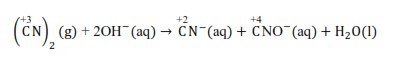It can be easily observed that the same compound$\left(^{+3} \mathrm{C} \mathrm{N}\right)_{2}$

is being reduced in

$\stackrel{+2}{\mathrm{C}} \mathrm{N}^{-}$and oxidized in${ }^{+4} \mathrm{C} \mathrm{NO}^{-}$simultaneously in the given equation. So it is a disproportionation reaction. Thus, it can be said that the alkaline decomposition of cyanogen is an example of a disproportionation reaction.

Question 21. The $\mathrm{Mn}^{3+}$ ion is unstable in solution and undergoes disproportionation to give $\mathrm{Mn}^{2+}, \mathrm{MnO}_{2}$, and $\mathrm{H}^{+}$ ion. Write a balanced ionic equation for the reaction.

Solution: The given reaction can be represented as:

$\mathrm{Mn}^{3+}(\mathrm{aq}) \rightarrow \mathrm{Mn}^{2+}(\mathrm{aq})$

$+\mathrm{MnO}_{2}(\mathrm{~s})+\mathrm{H}^{+}(\mathrm{aq})$

The oxidation half equation is:

$\mathrm{Mn}^{3+}(\mathrm{aq}) \rightarrow \mathrm{MnO}_{2}(\mathrm{~s})$

The 0 atoms and $\mathrm{H}^{+}$ ions are balanced by adding $2 \mathrm{H}_{2} 0$ molecules as:

$\mathrm{Mn}^{3+}+2 \mathrm{H}_{2} \mathrm{O}(\mathrm{l}) \rightarrow \mathrm{MnO}_{2}(\mathrm{~s})+4 \mathrm{H}^{+}(\mathrm{aq})$

To balance the charge add 1 electron in RHS

$\mathrm{Mn}^{3+}+2 \mathrm{H}_{2} \mathrm{O}(\mathrm{l}) \rightarrow \mathrm{MnO}_{2}(\mathrm{~s})$

$+4 \mathrm{H}^{+}(\mathrm{aq})+\mathrm{e}^{-} \ldots(\mathrm{i})$

The reduction half equation is:

$\mathrm{Mn}^{3+}(\mathrm{aq}) \rightarrow \mathrm{Mn}^{+2}(\mathrm{aq})$

To balance the charge add 1 electron in LHS

$\mathrm{Mn}^{3+}(\mathrm{aq})+\mathrm{e}^{-} \rightarrow \mathrm{Mn}^{2+}(\mathrm{aq}) \ldots(\mathrm{ii})$

The balanced chemical equation can be obtained by adding equation (i) and (ii) as:

$2 \mathrm{Mn}^{3+}(\mathrm{aq})+2 \mathrm{H}_{2} \mathrm{O}(\mathrm{l}) \rightarrow \mathrm{MnO}_{2}(\mathrm{~s})$

$+2 \mathrm{Mn}^{2+}(\mathrm{aq})+4 \mathrm{H}^{+}(\mathrm{aq})$

Question 22. Consider the elements :

Cs, Ne, I and F

(a) Identify the element that exhibits only negative oxidation state.

(b) Identify the element that exhibits only positive oxidation state.

(c) Identify the element that exhibits both positive and negative oxidation states.

(d) Identify the element which exhibits neither the negative nor does the positive oxidation state.

Solution: (a) $\mathrm{F}$ exhibits only negative oxidation state of $-1$ because it has highest electronegativity

(b) Cs exhibits positive oxidation state of $+1$ because it is alkali metal atom

(c) I exhibit both positive and negative oxidation states. It exhibits oxidation states of $-1,+1,+3,+5$, and $+7$

(d) The oxidation state of Ne is zero. It can not exhibits negative or positive oxidation states because it has complete octate

Question 23. Chlorine is used to purify drinking water. Excess of chlorine is harmful. The excess of chlorine is removed by treating with sulphur dioxide. Present a balanced equation for this redox change taking place in water.

Solution: The given redox reaction can be represented as:

$\mathrm{Cl}_{2}(\mathrm{~s})+\mathrm{SO}_{2}(\mathrm{aq})+\mathrm{H}_{2} \mathrm{O}(\mathrm{l})$

$\rightarrow \mathrm{Cl}^{-}(\mathrm{aq})+\mathrm{SO}_{4}^{2-}$

The oxidation half-reaction is.

$\mathrm{SO}_{2}(\mathrm{aq}) \rightarrow \mathrm{SO}_{4}^{2-}(\mathrm{aq})$

to balance the $0-$ atom add $\mathrm{H}_{2} \mathrm{O}$ in oxygen deficient side

$2 \mathrm{H}_{2} \mathrm{O}(\mathrm{l})+\mathrm{SO}_{2}(\mathrm{aq}) \rightarrow \mathrm{SO}_{4}^{2-}(\mathrm{aq})$

to balance the $\mathrm{H}$ - atom add $\mathrm{H}^{+}$ in hydrogen deficient side

$2 \mathrm{H}_{2} \mathrm{O}(\mathrm{l})+\mathrm{SO}_{2}(\mathrm{aq})$’

$\rightarrow \mathrm{SO}_{4}^{2-}(\mathrm{aq})+4 \mathrm{H}^{+}(\mathrm{aq})$

$2 \mathrm{H}_{2} \mathrm{O}(\mathrm{l})+\mathrm{SO}_{2}(\mathrm{aq}) \rightarrow \mathrm{SO}_{4}^{2-}(\mathrm{aq})$

$+4 \mathrm{H}^{+}(\mathrm{aq})+2 \mathrm{e}^{-}$

$\mathrm{Cl}_{2}(\mathrm{~s}) \rightarrow \mathrm{Cl}^{-}(\mathrm{aq})$

The chlorine atoms are balanced as:

$\mathrm{Cl}_{2}(\mathrm{~s}) \rightarrow 2 \mathrm{Cl}^{-}(\mathrm{aq})$

$2 \mathrm{e}^{-}+\mathrm{Cl}_{2}(\mathrm{~s}) \rightarrow 2 \mathrm{Cl}^{-}(\mathrm{aq})$

Add both half reaction we get net reaction :

$\mathrm{Cl}_{2}(\mathrm{~s})+\mathrm{SO}_{2}(\mathrm{aq})+2 \mathrm{H}_{2} \mathrm{O}(\mathrm{l}) \rightarrow 2 \mathrm{Cl}^{-}(\mathrm{aq})$

$+\mathrm{SO}_{4}^{2-}(\mathrm{aq})+4 \mathrm{H}^{+}(\mathrm{aq})$

Question 24. Refer to the periodic table given in your book and now answer the following questions:

(a) Select the possible non metals that can show disproportionation reaction.

(b) Select three metals that can show disproportionation reaction.

Solution: In disproportionation reactions, one of the reacting substances always contains an

element that can exist in at least three oxidation states,

(a) P, Cl, Br,I, and S can show disproportionation reactions as these elements can exist in three or more oxidation states,

(b) Mn, Cu, and Ga can show disproportionation reactions as these elements can exist in three or more oxidation states.

Question 25. In Ostwald's process for the manufacture of nitric acid, the first step involves the oxidation of ammonia gas by oxygen gas to give nitric oxide gas and steam. What is the maximum weight of nitric oxide that can be obtained starting only with $10.00 \mathrm{~g} .$ of ammonia and $20.00 \mathrm{~g}$ of oxygen?

Solution: The balanced chemical equation for the given reaction is given as:

$4 \mathrm{NH}_{3}+5 \mathrm{O}_{2} \rightarrow 4 \mathrm{NO}+6 \mathrm{H}_{2} \mathrm{O}$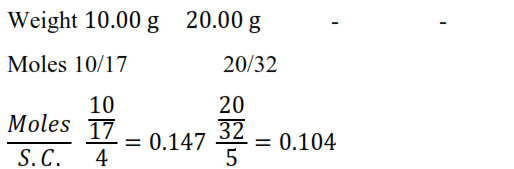$\mathrm{O}_{2}$ is limiting reagent

Thus, $68 \mathrm{~g}$ of $\mathrm{NH}_{3}$ reacts with $160 \mathrm{~g}$ of $\mathrm{O}_{2}$

Therefore, $10 \mathrm{~g}$ of $\mathrm{NH}_{3}$ reacts with $\frac{160 \times 10}{68}$ of $\mathrm{O}_{2}$, or $23.53 \mathrm{~g}$ of $\mathrm{O}_{2}$.

Therefore, $O_{2}$ is the limiting reagent (we have considered the amount of $0_{2}$ to calculate the weight of nitric oxide obtained in the reaction).

Now, $160 \mathrm{~g}$ of $\mathrm{O}_{2}$ gives $120 \mathrm{~g}$ of $\mathrm{NO}$.

Therefore, $20 \mathrm{~g}$ of $\mathrm{O}_{2}$ gives $\frac{120 \times 20}{160} \mathrm{~g}$ of $\mathrm{N}$, or $15 \mathrm{~g}$ of $\mathrm{NO}$.

Hence, a maximum of $15 \mathrm{~g}$ of nitric oxide can be obtained.

Question 26. Using the standard electrode potentials given in the Table $8.1$, predict if the reaction between the following is feasible:

(a) $\mathrm{Fe}^{3+}(\mathrm{aq})$ and $\mathrm{I}^{-}(\mathrm{aq})$

(b) $\mathrm{Ag}^{+}(\mathrm{aq})$ and $\mathrm{Cu}(\mathrm{s})$

(c) $\mathrm{Fe}^{3+}(\mathrm{aq})$ and $\mathrm{Cu}(\mathrm{s})$

(d) $\mathrm{Ag}(\mathrm{s})$ and $\mathrm{Fe}^{3+}(\mathrm{aq})$

(e) $\mathrm{Br}_{2}(\mathrm{aq})$ and $\mathrm{Fe}^{2+}(\mathrm{aq})$

Solution: (a) The possible reaction between $\mathrm{Fe}^{3+}(\mathrm{aq})+\mathrm{I}^{-}(\mathrm{aq})$ is given by

$2 \mathrm{Fe}^{3+}(\mathrm{aq})+\mathrm{I}^{-}(\mathrm{aq})$

$\rightarrow 2 \mathrm{Fe}^{2+}(\mathrm{aq})+\mathrm{I}_{2}(\mathrm{~s})$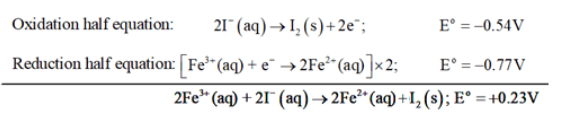$\mathrm{E}^{\mathrm{o}}$ for the overall reaction is positive. Thus, the reaction between

$\mathrm{Fe}^{3+}(\mathrm{aq})+\mathrm{I}^{-}(\mathrm{aq})$ is feasible.

(b) The possible reaction between $\mathrm{Ag}^{+}(\mathrm{aq})+\mathrm{Cu}(\mathrm{s})$ is given by,

$2 \mathrm{Ag}^{+}(\mathrm{aq})+\mathrm{Cu}(\mathrm{s}) \rightarrow 2 \mathrm{Ag}(\mathrm{s})+\mathrm{Cu}^{2+}(\mathrm{aq})$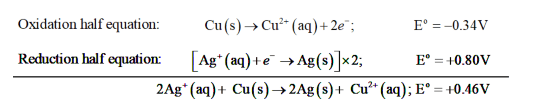$\mathrm{E}^{\mathrm{o}}$ for the overall reaction is positive. Thus, the reaction between $\mathrm{Ag}^{+}(\mathrm{aq})$ and $\mathrm{Cu}(\mathrm{s})$ is feasible.

(c) The possible reaction between $\mathrm{Fe}^{3+}$ (aq) and $\mathrm{Cu}(\mathrm{s})$ is given by,

$2 \mathrm{Fe}^{3+}(\mathrm{aq})+\mathrm{Cu}(\mathrm{s}) \rightarrow 2 \mathrm{Fe}^{2+}(\mathrm{s})+\mathrm{Cu}^{2+}(\mathrm{aq})$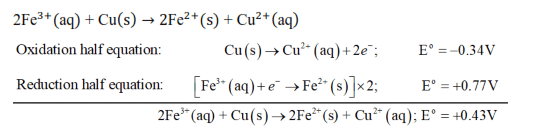$\mathrm{E}^{\circ}$ for the overall reaction is positive. Thus, the reaction between $\mathrm{Fe}^{3+}(\mathrm{aq})$ and $\mathrm{Cu}(\mathrm{s})$ is feasible.

(d) The possible reaction between $\mathrm{Fe}^{3+}(\mathrm{aq})$ and $\mathrm{Ag}(\mathrm{s})$ is given by,

$\mathrm{Ag}(\mathrm{s}) 2 \mathrm{Fe}^{3+}(\mathrm{aq}) \rightarrow \mathrm{Ag}^{+}(\mathrm{aq})+\mathrm{Fe}^{2+}(\mathrm{aq})$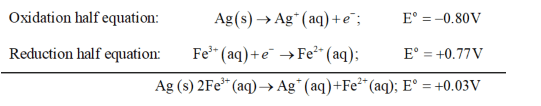Here $E^{o}$ for the overall reaction is positive. Thus, the reaction between $\mathrm{Fe}^{3+}(\mathrm{aq})$ and $\mathrm{Ag}(\mathrm{s})$ is not feasible.

(e) The possible reaction between $\mathrm{Br}_{2}(\mathrm{aq})$ and $\mathrm{Fe}^{2+}(\mathrm{aq})$ is given by

$\mathrm{Br}_{2}(\mathrm{aq})+2 \mathrm{Fe}^{2+}(\mathrm{aq}) \rightarrow 2 \mathrm{Br}^{-}$

$+2 \mathrm{Fe}^{3+}(\mathrm{aq})$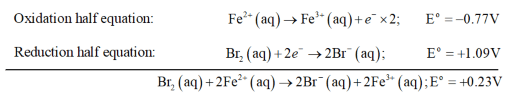Here $\mathrm{E}^{\circ}$ for the overall reaction is positive. Thus, the reaction between $\mathrm{Br}_{2}(\mathrm{aq})$ and $\mathrm{Fe}^{2+}(\mathrm{aq})$ is feasible.

Question 27. Predict the products of electrolysis in each of the following:

(i) An aqueous solution of $\mathrm{AgNO}_{3}$ with silver electrodes

(ii) An aqueous solution $\mathrm{AgNO}_{3}$ with platinum electrodes

(iii) A dilute solution of $\mathrm{H}_{2} \mathrm{SO}_{4}$ with platinum electrodes

(iv) An aqueous solution of $\mathrm{CuCl}_{2}$ with platinum electrodes.

Solution: (i) $\mathrm{AgNO}_{3}$ ionizes in aqueous solutions to form $\mathrm{Ag}^{+}$ and $\mathrm{NO}_{3}^{-}$ ions. On electrolysis,either $\mathrm{Ag}^{+}$ ions or $\mathrm{H}_{2} \mathrm{O}$ molecules can be reduced at the cathode. But the reduction potential of $\mathrm{Ag}^{+}$ ions is higher than that of $\mathrm{H}_{2} \mathrm{O}$.

$\mathrm{Ag}^{+}(\mathrm{aq})+\mathrm{e}^{-} \rightarrow \mathrm{Ag}(\mathrm{s}) ; \mathrm{E}^{\circ}=-0.80 \mathrm{~V}$

$\left.2 \mathrm{H}_{2} \mathrm{O}(\mathrm{l})+2 \mathrm{e}^{-} \rightarrow \mathrm{H}_{2} \mathrm{~g}\right)+20 \mathrm{H}-(\mathrm{aq}) ; \mathrm{E}^{\mathrm{o}}=0.83 \mathrm{~V}$

Therefore, Ag metal gets oxidized at the anode.

(ii) Pt cannot be oxidized easily. Hence, at the anode, oxidation of water occurs to liberate $\mathrm{O}_{2}$.

At the cathode, Ag + ions are reduced and get deposited.

(iii) $\mathrm{H}_{2} \mathrm{SO}_{4}$ ionizes in aqueous solutions to give $\mathrm{H}^{+}$ and $\mathrm{SO}_{4}^{2-}$ ions.

$\mathrm{H}_{2} \mathrm{SO}_{4}(\mathrm{aq}) \rightarrow 2 \mathrm{H}^{+}(\mathrm{aq})+\mathrm{SO}_{4}^{2-}$

On electrolysis, either of $\mathrm{H}^{+}$ ions or $\mathrm{H}_{2} \mathrm{O}$ molecules can get reduced at the cathode. But the reduction potential of $\mathrm{H}^{+}$ ions is higher than that of $\mathrm{H}_{2} \mathrm{O}$ molecules.

$2 \mathrm{H}^{+}(\mathrm{aq})+2 \mathrm{e}^{-} \rightarrow \mathrm{H}_{2}(\mathrm{~g}) ; \mathrm{E}^{\circ}=0.0 \mathrm{~V}$

$2 \mathrm{H}_{2} \mathrm{O}(\mathrm{aq})+2 \mathrm{e}^{-} \rightarrow \mathrm{H}_{2}(\mathrm{~g}) \mathrm{OH}^{-}(\mathrm{aq}) ; \mathrm{E}^{\circ}=-0.83 \mathrm{~V}$

Hence, at the cathode, $\mathrm{H}^{+}$ ions are reduced to liberate $\mathrm{H}_{2}$ gas.

On the other hand, at the anode, either of $\mathrm{SO}_{4}^{2-}$ ions or $\mathrm{H}_{2} \mathrm{O}$ molecules can get oxidized. But the oxidation of $\mathrm{SO}_{4}^{2-}$ involves breaking of more bonds than that of $\mathrm{H}_{2} \mathrm{O}$ molecules. Hence, $\mathrm{SO}_{4}^{2-}$ ions have a lower oxidation potential than $\mathrm{H}_{2} \mathrm{O}$. Thus, $\mathrm{H}_{2} \mathrm{O}$ is oxidized at the anode to liberate $\mathrm{O}_{2}$ molecules.

(iv) In aqueous solutions, $\mathrm{CuCl}_{2}$ ionizes to give $\mathrm{Cu}^{2+}$ and $\mathrm{Cl}^{-}$ ions as:

$\mathrm{CuCl}_{2}(\mathrm{aq}) \rightarrow \mathrm{Cu}^{2+}(\mathrm{aq})+2 \mathrm{Cl}^{-}(\mathrm{aq})$

On electrolysis, either of $\mathrm{Cu}^{2+}$ ions or $\mathrm{H}_{2} \mathrm{O}$ molecules can get reduced at the cathode. But the reduction potential of $\mathrm{Cu}^{2+}$ is more than that of $\mathrm{H}_{2} \mathrm{O}$ molecules.

$\mathrm{Cu}^{2+}(\mathrm{aq})+2 \mathrm{e}^{-} \rightarrow \mathrm{Cu}(\mathrm{aq}) ; \mathrm{E}^{\circ}=+0.34 \mathrm{~V}$

$\mathrm{H}_{2} \mathrm{O}(\mathrm{l})+2 \mathrm{e}^{-} \rightarrow \mathrm{H}_{2}(\mathrm{~g})+2 \mathrm{OH}^{-} ; \mathrm{E}^{\mathrm{o}}=-0.83 \mathrm{~V}$

Hence, $\mathrm{Cu}^{2+}$ ions are reduced at the cathode and get deposited.

Similarly, at the anode, either of $\mathrm{Cl}^{-}$ or $\mathrm{H} 2 \mathrm{O}$ is oxidized. The oxidation potential of $\mathrm{H} 2 \mathrm{O}$ is

higher than that of $\mathrm{Cl}^{-}$

$2 \mathrm{Cl}^{-}(\mathrm{aq}) \rightarrow \mathrm{Cl}_{2}(\mathrm{~g})+2 \mathrm{e}^{-} ; \mathrm{E}^{0}=-1.36 \mathrm{~V}$

$2 \mathrm{H}_{2} \mathrm{O}(\mathrm{l}) \rightarrow \mathrm{O}_{2}(\mathrm{~g})+4 \mathrm{H}^{+}(\mathrm{aq})+4 \mathrm{e}^{-} ; \mathrm{E}^{0}=-1.23 \mathrm{~V}$

But oxidation of $\mathrm{H}_{2} \mathrm{O}$ molecules occurs at a lower electrode potential than that of $\mathrm{Cl}^{-}$ ions because of over-voltage (extra voltage required to liberate gas). As a result, $\mathrm{Cl}^{-}$. ions are oxidized at the anode to liberate $\mathrm{Cl}_{2}$ gas.

Similarly, at the anode, either of $\mathrm{Cl}^{-}$ or $\mathrm{H}_{2} \mathrm{O}$ is oxidized. The oxidation potential of $\mathrm{H}_{2} \mathrm{O}$ is higher than that of $\mathrm{Cl}^{-}$.

Question 28. Arrange the following metals in the order in which they displace each other from the solution of their salts.

$\mathrm{Al}, \mathrm{Cu}, \mathrm{Fe}, \mathrm{Mg}$ and $\mathrm{Zn}$

Solution: A metal of stronger reducing power displaces another metal of weaker reducing nower from its solution of salt.

According to electrochemical series the order of the increasing reducing the power of the given metals is $\mathrm{Cu}<\mathrm{Fe}<\mathrm{Zn}<\mathrm{Al}<\mathrm{Mg}$

Hence, we can say that Mg can displace Al from its salt solution, but Al cannot displace Mg.

Thus, the order in which the given metals displace each other from the solution of their salts is given below: $\mathrm{Mg}>\mathrm{Al}>\mathrm{Zn}>\mathrm{Fe},>\mathrm{Cu}$

Question 29. Given the standard electrode potentials,

$\mathrm{K}^{+} / \mathrm{K}=-2.93 \mathrm{~V}$

$\mathrm{Ag}^{+} / \mathrm{Ag}=0.80 \mathrm{~V}$

$\mathrm{Hg}^{2+} / \mathrm{Hg}=0.79 \mathrm{~V}$

$\mathrm{Mg}^{2+} / \mathrm{Mg}=-2.37 \mathrm{~V}$

$\mathrm{Cr}^{3+} / \mathrm{Cr}=-0.74 \mathrm{~V}$

arrange these metals in their increasing order of reducing power.

Solution: The lower the electrode potential, the stronger is the reducing agent. Therefore, the increasing order of the reducing power of the given metals is $\mathrm{Ag}<\mathrm{Hg}<\mathrm{Cr}<$ $\mathrm{Mg}<\mathrm{K}$

Question 30. Depict the galvanic cell in which the reaction $\mathrm{Zn}(\mathrm{s})+2 \mathrm{Ag}^{+}(\mathrm{aq}) \rightarrow \mathrm{Zn}^{2+}(\mathrm{aq})+$

$2 \mathrm{Ag}(\mathrm{s})$ takes place, Further show:

(i) which of the electrode is negatively charged,

(ii) the carriers of the current in the cell, and

(iii) individual reaction at each electrode

Solution: The galvanic cell corresponding to the given redox reaction can be represented as:

$\mathrm{Zn}\left|\mathrm{Zn}^{2+}(\mathrm{aq})\right| \mathrm{Ag}^{+}(\mathrm{aq}) \mid \mathrm{Ag}$

(i) Zn electrode is negatively charged because at this electrode, Zn oxidizes to $\mathrm{Zn}^{2+}$ and the leaving electrons accumulate on this electrode.

(ii) Ions are the carriers of current in the cell.

(iii) The reaction taking place at the $\mathrm{Zn}$ electrode can be represented as: $\mathrm{Zn}(\mathrm{s}) \rightarrow \mathrm{Zn}^{2+}(\mathrm{aq})+2 \mathrm{e}^{-}$

And the reaction taking place at the Ag electrode can be represented as:

$\mathrm{Ag}^{+}(\mathrm{aq})+\mathrm{e}^{-} \rightarrow \mathrm{Ag}(\mathrm{s})$